### Choose language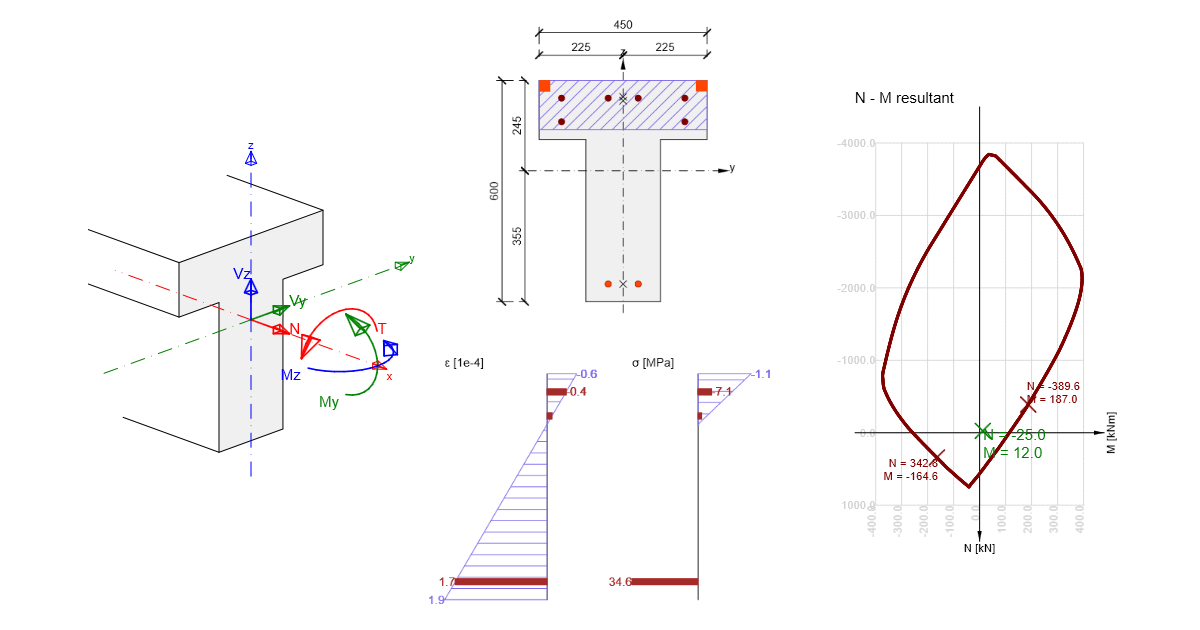# IDEA StatiCa RCS – Structural design of 1D concrete members

$$Design of reinforced concrete sections according to EN 1992-1-1 and EN 1992-2.Bending Shear Torsion Interaction Stress limitation check Crack control N-M-κ diagram Literature Bending Methods for sectional capacity checkTwo well-known methods can be used to check the ultimate limit state for 1D concrete members. The first one will give us the cross-sectional ultimate strength in the form of an interaction area or an interaction diagram (in the case of bending moment in one direction). Cross-sectional capacity can be determined as the ratio of acting internal forces to limit state forces. The second one is finding equilibrium in a cross-section, where we are looking for the actual behaviour of the loaded section, the use of materials in terms of stresses, and insight into the vulnerabilities of the section.General design assumptions and calculation assumptions for Ultimate Limit State Strain ε in the reinforcement and concrete shall be assumed directly proportional to the distance from the neutral axis (plane sections remain plane). Interaction of the reinforcement and concrete is ensured by concrete and reinforcement interaction without slip (strain ε supports the strain in concrete adjacent fibers are the same). The tensile strength of concrete is neglected (all tensile stresses are transmitted by the reinforcement). Concrete compression stresses in the compression zone are calculated in relation to the strain calculated from stress-strain diagrams. Reinforcement stresses are calculated in relation to the strain from stress-strain diagrams. Compressive concrete strain with an ultimate strain limit εcu2 (Parabola-rectangle diagram for concrete under compression) and εcu3 (Bi-linear stress-strain relation), . The compressive strain of reinforcement is without limitation in the case of the horizontal plastic top branch, in the case of the inclined plastic top branch the strain is limited εud,. A limit state is considered when the state of at least one of the materials exceeds the ultimate limit strain (if εu is not limited, the compressed concrete is governing).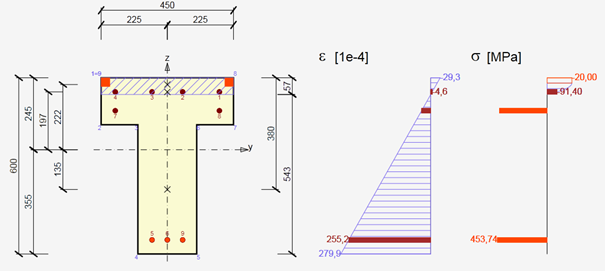$\textsf{\textit{\footnotesize{\qquad Strain stress.}}}$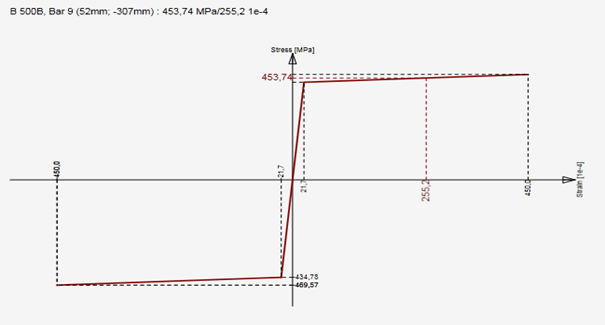$\textsf{\textit{\footnotesize{\qquad Stress-strain design diagram for reinforcing steel with inclined top branch.}}}$Interaction diagramThe first option is to check the cross-section by an interaction surface (or interaction diagram). An explanation is provided on a sample of the interaction surfaces for the reinforced square section from the example in the figure below. On the interaction surface are located points defining the ultimate limit state of the examined cross-section. The interaction surface is drawn from the points (N, My, Mz), which are determined by stress integration in the cross-section, which has achieved ultimate limit strain in one of the materials. For a 3D interaction, the surface can be derived from a 2D interaction diagram, which is a closed curve, which corresponds to the stress of a constantly rotated neutral axis.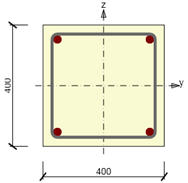$\textsf{\textit{\footnotesize{\qquad Symmetrical reinforced cross-section.}}}$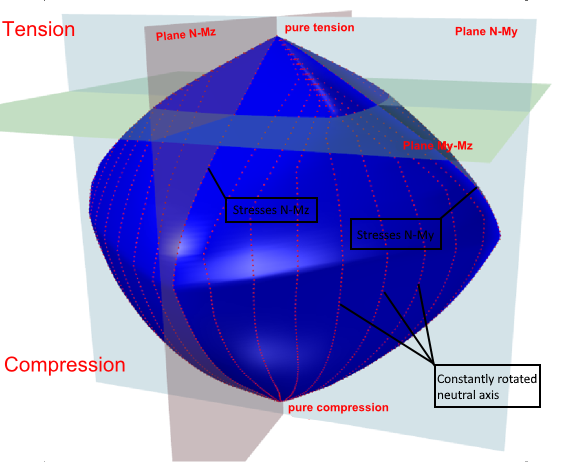$\textsf{\textit{\footnotesize{\qquad Interaction surface shows failure conditions for all load cases of normal force and bending moments.}}}$For the case of a symmetrical cross-section about the y-axis, the interaction diagram is symmetrical around the plane N-My. Identically, for the case of a symmetrical cross-section about the z-axis, the interaction diagram is symmetrical around plane N-My. The one-sided reinforced section introduces a flattened shape of the interaction diagram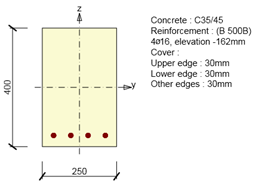$\textsf{\textit{\footnotesize{\qquad Single symmetrical reinforced cross-section.}}}$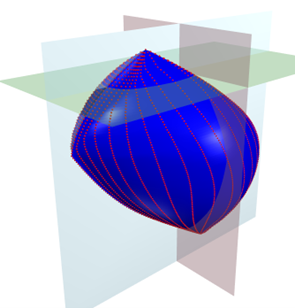$\textsf{\textit{\footnotesize{\qquad Interaction surface for cross-section with single symmetric reinforcement.}}}$The points defining the ultimate limit state are received from stress integration. The figure below displays strain at the ultimate limit state.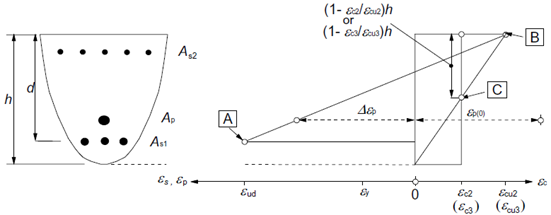$\textsf{\textit{\footnotesize{\qquad Strain distributions at the ultimate limit state (taken from ).}}}$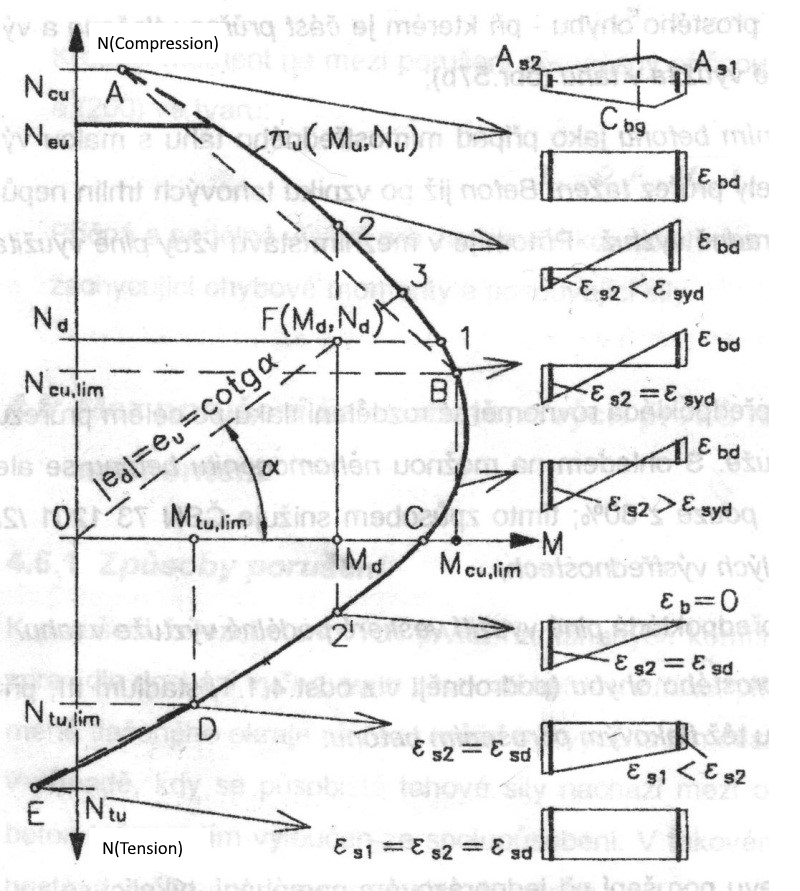The interaction diagram shows cross-section failure under normal force and bending moments. Respecting the 2D diagram problem (closed curve laying on interaction surface) we can find out the strain plane is passing through the neutral axis and critical point [y, z, ε], which is considered as critical point R. Point [y, z] defines a point in cross-section with the value of strain ε at the ultimate limit state. The neutral axis inclination is constant for all points of the 2D diagram.In case the compressive stress in concrete is critical for design, point R is matching the farthest compressed concrete fiber or to limiting point C. However, this can be applied only if that section is made from one type of concrete - not such as a mixed cross-section. In the case the tensile stress in reinforcement is critical for design (strain εud is exceeded at the ultimate limit state for one or more bars), there must be fulfilled condition that for the given strain plane the value εud is not exceeded at any other bar.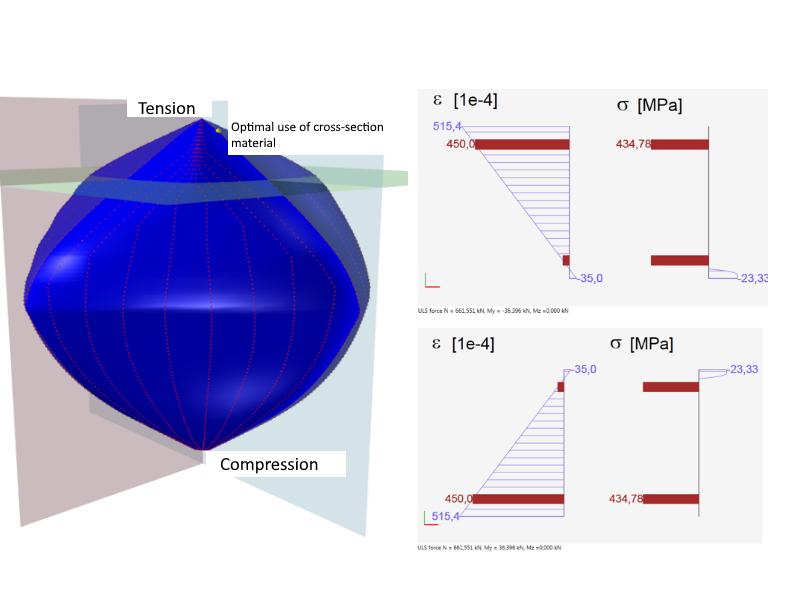$\textsf{\textit{\footnotesize{\qquad Optimal use of cross-section material.}}}$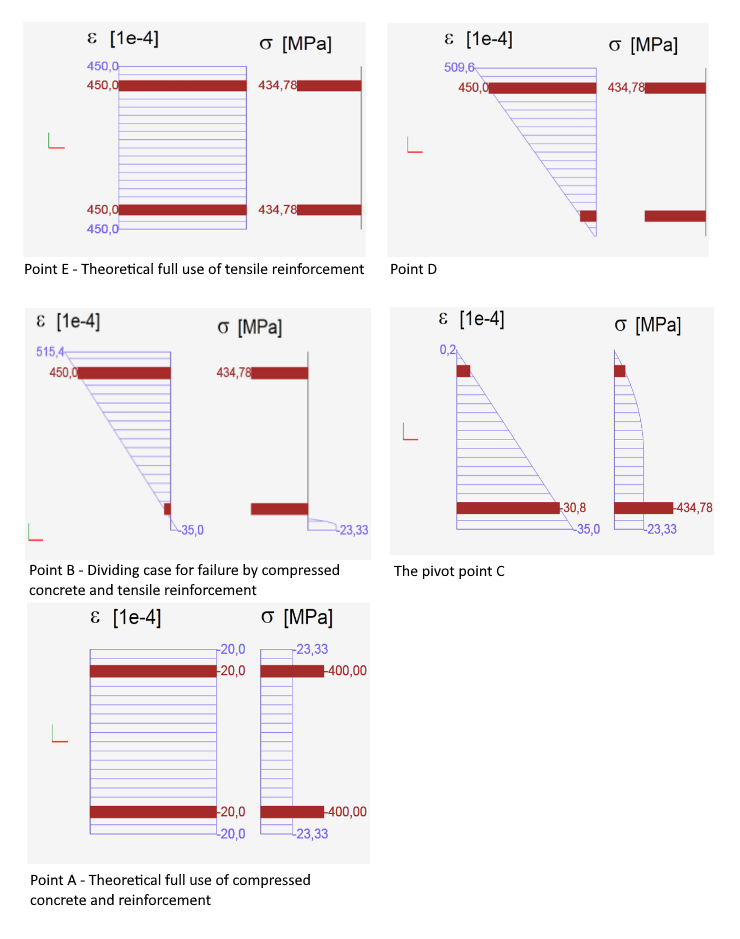$\textsf{\textit{\footnotesize{\qquad Characteristic strain plane positions calculated for purpose of interaction diagram.}}}$The picture above shows that the diagram can be divided into two parts: the part where the failure is caused by tensile force and the part which fails by a compressive force. The limit points correspond to the case above, where the extreme inclination of the strain plane also can be seen. When drawing an interaction diagram the plane strain inclination of a cross-section is changing in this interval, while we search for the point R (see above). Based on that defined plane we perform the integration to get the stress at the ultimate limit state.Cross-section subjected to axial force and bending moment checkThe check of a cross-section subjected to axial force and bending moment relies in proving that the checked stresses (combination Nd, Mzd, Mzd) are located inside or on the surface interaction area. Different methods can do this. The following example demonstrates the check of a rectangular cross-section subjected to forces Nd = -500 kN, Myd = 120 kNm, Mzd = 100 kNm.Method NuMuMuTo define the resistance of a cross-section we assume proportional changes in all of the internal force components (the eccentricity of the normal force remains constant) until the interactive surface has been developed. The change of involved internal forces can be interpreted as moving along a line connecting the start coordinate system (0,0,0) and the point defined by the internal forces (NEd, MEd,y, MEd,z). The two intersections of this line with the interaction surface, which can be found, represents two sets of forces at the ultimate limit state. At each intersection, the program determines three forces at the limit state: the design axial force resistance NRd and the corresponding design moments of resistance MRdy, MRdz.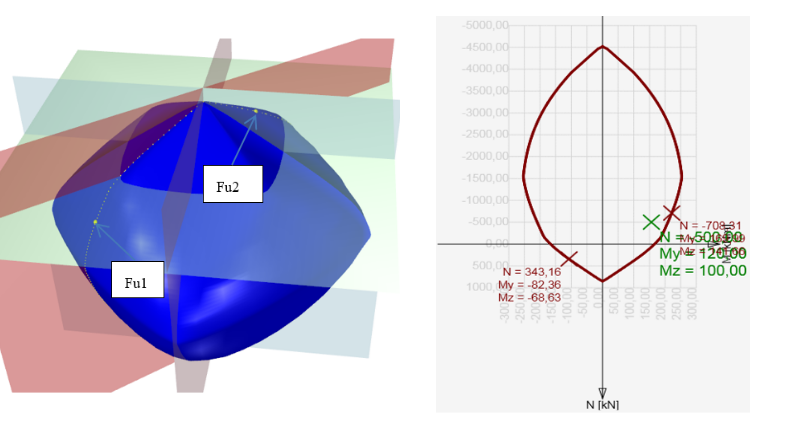Method NuMMTo define the resistance of cross-section we assume constant normal force (which is equal to the acting design normal force) and proportional changes in bending moments until the interactive surface has been developed. The change of involved internal forces can be interpreted as moving in a horizontal plane along the line connecting the point (NEd,0,0) and the point defined by the acting internal forces (NEd, MEd,y, MEd,z). The two intersections of this line with the interaction surface, which can be found, represents two sets of forces at the ultimate limit state. At each intersection the program determines three forces at the limit state: the design resisting moments MRdy, MRdz and (corresponding) acting design normal force NEd.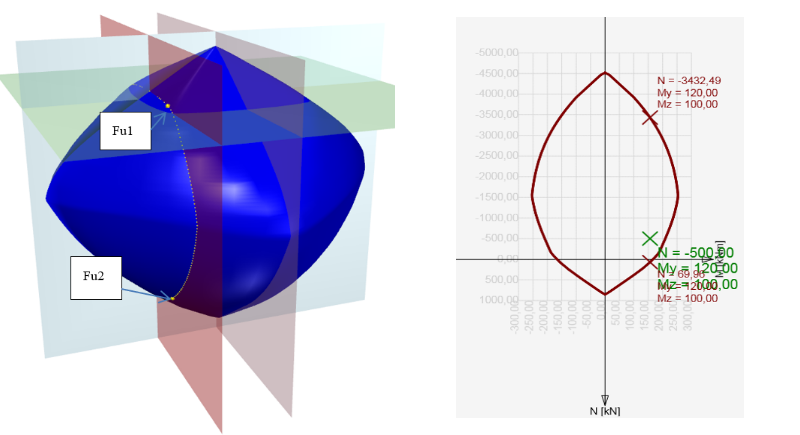Method NMuMuTo define the resistance of the cross-section we assume a constant normal force (which is equal to the acting design normal force) and proportional changes in bending moments until the interactive surface has been developed. The change of the involved internal forces can be interpreted as moving in a horizontal plane along the line connecting the point (NEd,0,0) and the point defined by the acting internal forces (NEd, MEd,y, MEd,z). The two intersections of this line with the interaction surface, which can be found, represent two sets of forces at the ultimate limit state. At each intersection, the program determines three forces at the limit state: the design resisting moments MRdy, MRdz, and (corresponding) acting design normal force NEd.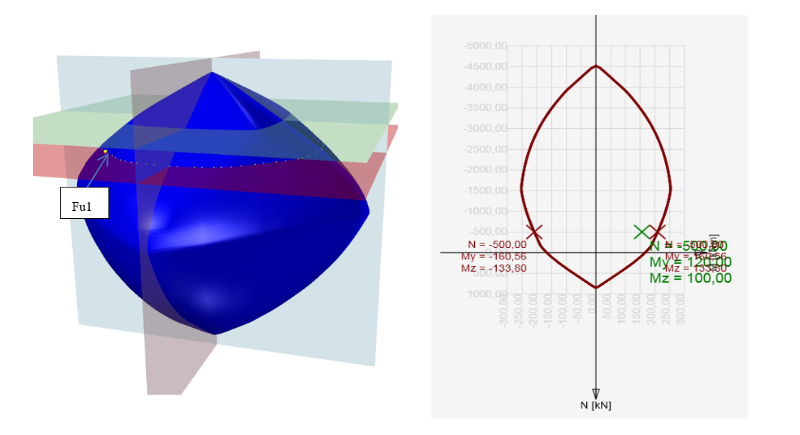Finding the section responseAnother possibility to check the cross-section is by finding the cross-section response (i.e. Strain and stress distribution from acting internal forces). This method is also known as the method of limit deformation. The level of acting stresses in each fiber (in the case of plane bending in each layer) in each reinforced bar is calculated depending on the strain of the Stress-strain diagram of the material. Finding the cross-section response is calculated using the numerical method specified in . The principle consists of the gradual load increment of the section by the unbalanced components of not-transferred forces. Those are obtained by integrating the stress over the section using Stress-strain diagrams. If the stress value can be found for the strain in the Stress-strain diagram, see Figure below (a), the calculated stress is correct assuming linear elastic material. In cases (b) and(c), the stress for a linear calculation reaches unrealistic values, and part (b) or entire value (c) cannot be transmitted by the material. Integrating not-transferred stresses we get not-transferred internal forces, and their resultants should be added to the internal forces of variable loads.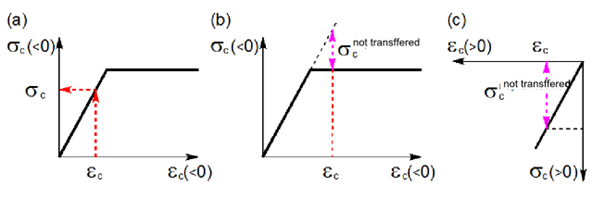Not-transferred stresses in Stress-strain diagrams.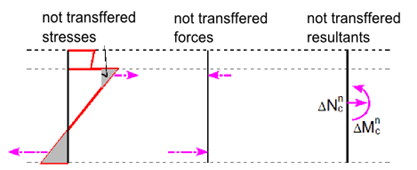Not-transferred inner forces. This calculation method requires the use of numerical methods for integrating the stress over the cross-section area and for nonlinear analysis of equilibrium equations in the section. Iteration is terminated at the time when the convergence criteria are met.$\frac{{{F_e} - {F_i}}}{{{F_e}}} \le max\left\{ {e,d} \right\}$where Fe is section load,Fi is section response (internal forces calculated on the base of the strain plane).If a is the approximate (approximated) value and b is the exact (true) value, then absolute deviation is given by the following equation.$e = \left| {b - a} \right|$Relative deviation is given by the following formula:$d = \left| {\frac{{b - a}}{b}} \right|$In most programs, you can set these convergence criteria (default values are 1% as relative error, 100 N, 100 Nm as the absolute error of normal force and moments). So if we have the input of N = 0 kN, My = 100 kNm, Mz = 0 kNm and integrated forces after iteration N = - 0.07 kN, My = 100.5 kNm, Mz = 0.02 kNm, the evaluation will be as follows. With respecting the N and Mz being equal to 0, a comparison with absolute deviation can be done:The value of normal force 100N> | 70 | N The value of the bending moment Mz 100Nm> | 20 | Nm The value of the bending moment My$d = \left| {\frac{{b - a}}{b}} \right| = \frac{{100 - 100,5}}{{100}} = 0,005\; < 0,01$Cross-section check by the responseIn the case of finding a balance in the cross-section, plane strain is known. From the plane strain we can calculate strain anywhere in the section, then the stress or inner forces in reinforcement bars, cross-section or its parts using Stress-strain diagrams of the materials. The calculated stress and strain values we compare with the limit strain value from the stress-strain diagrams of the used materials. The advantage of this method is that we get a complete image of the stress and strain values in the section of the internal forces acting on the cross-section. Shear With respect to fragile failure the shear check is one of the important checks of a reinforced concrete section.Calculation procedureCalculation of shear resistance is composed of several basic parts. At first we should analyze whether cracks due to bending occur or not in the checked location. If any, use the calculation according to EN 1992-1-1 , Article 6.2.2 (1). Otherwise, we determine whether it is plane concrete or poorly reinforced concrete, then proceed in accordance with EN 1992-1-1 Article 12.6.3. For reinforced uncracked concrete (without shear reinforcement) we check according to EN 1992-1-1 Article 6.2.2 (2). For Elements, where is required shear reinforcement we check according to Article 6.2.3 .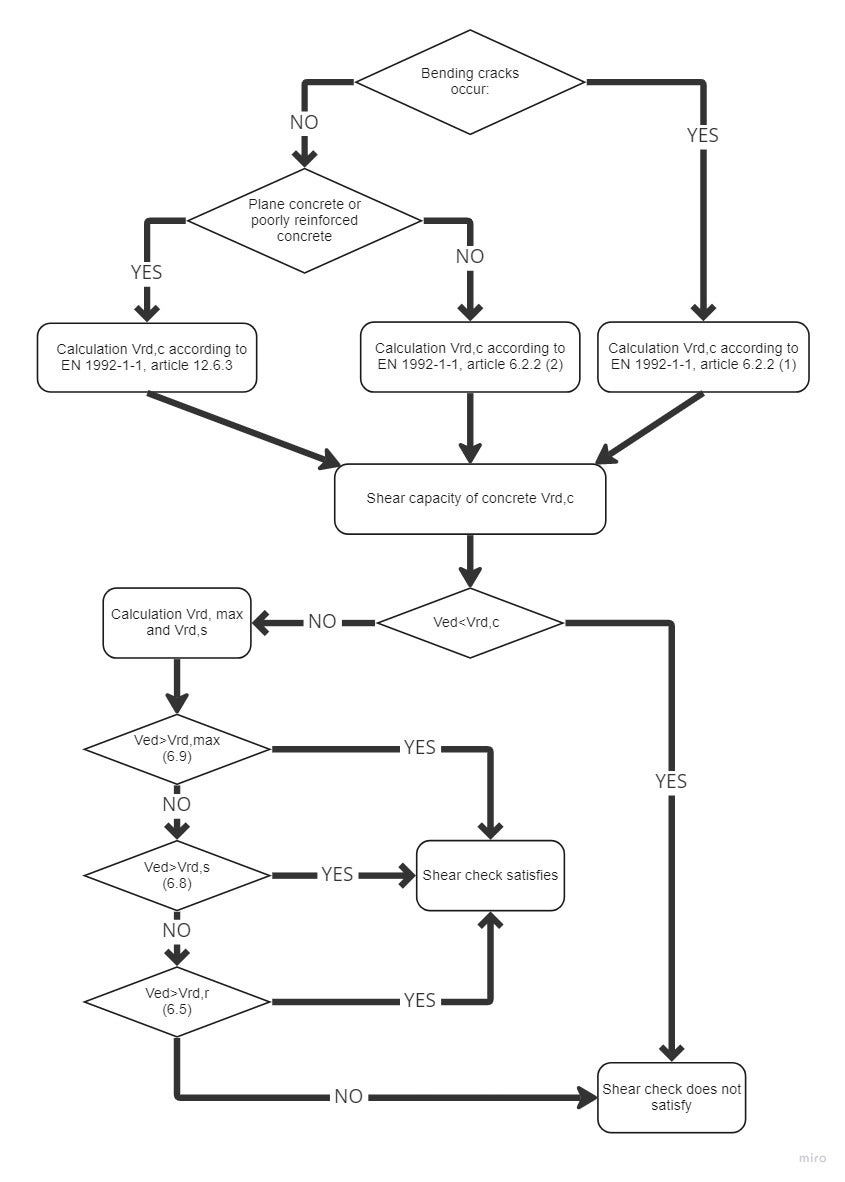$\textsf{\textit{\footnotesize{\qquad Process diagram for shear check.}}}$Shear resistance of members without shear reinforcementShear resistance of members in cracked bending zones (art. 6.2.2 (1) )Shear resistance of reinforced concrete members without shear reinforcement subject to bending moment is given by: ${{V}_{Rd,cm}}=~{{C}_{Rd.c}}k~{{\left( 100~{{\varrho }_{l}}{{f}_{ck}} \right)}^{{}^{1}/{}_{3}}}~{{b}_{w}}d$Which was defined on the base of tests executed on a representative number of simple beams in case of failure by shear force. Since the above resistance may be zero for elements without longitudinal reinforcement (rl), for poorly reinforced members was derived equations. Since the above resistance may be zero for members without longitudinal reinforcement (rl), for the poorly reinforced members was determined by equation${{V}_{Rd,c}}\ge ~{{\upsilon }_{min}}{{b}_{w}}d$For shear resistance with influence of normal force was determined by equation${{V}_{Rd,cn}}=~{{k}_{1}}{{\sigma }_{cp}}~{{b}_{w}}d$Shear resistance in its complete expression which is corresponding with EN 1992-1-1 art. 6.2.2 (1)${{V}_{Rd,c}}=~\left[ {{C}_{Rd.c}}k~{{\left( 100~{{\varrho }_{l}}{{f}_{ck}} \right)}^{{}^{1}/{}_{3}}}+{{k}_{1}}{{\sigma }_{cp}} \right]~{{b}_{w}}d$With minimum of${{V}_{Rd,c}}=~\left( {{\upsilon }_{min}}+{{k}_{1}}{{\sigma }_{cp}} \right){{b}_{w}}d$where CRd,c = 0,18 / γc,k cross-section height factor $k=1+\sqrt{\frac{200}{d}}<2,0$ρ1 reinforcement ratio for longitudinal reinforcement${{\varrho }_{l}}=\frac{{{A}_{sl}}}{{{b}_{w}}d}\le 0,02$fck characteristic compressive cylinder strength of concrete at 28 daysk1 = 0,15σcp = NEd / Ac < 0,2 fcd v MPabw smallest width of the cross-section in the tensile aread effective depth of a cross-sectionυmin minimal equivalent shear strength υmin = 0.035 k3/2 fck1/2Shear resistance of members in uncracked bending zones (art. 6.2.2 (2) )Shear resistance of members in uncracked bending zones can be determined from the Mohr circle. Into equation${{\sigma }_{1,2}}=\frac{{{\sigma }_{x}}+{{\sigma }_{y}}}{2}\pm \sqrt{{{\left( \frac{{{\sigma }_{x}}-{{\sigma }_{y}}}{2} \right)}^{2}}+\tau _{z}^{2}}$We substitute σx = σcp a τz = VRd,c S / (I bw) and figure out VRd,c and get equation corresponding with formula given in EN 1992-1-1 art. 6.2.2 (2)where I is the second moment of area,bw is the width of the cross-section at the centroidal axisS is the first moment of area above and about the centroidal axis,fctd design axial tensile strength of concrete in MPa, scp is the concrete compressive stress at the centroidal axis due to loading and/or prestressing,al transmission length factor, usually 1,0.In relation with the above it should be noted that in areas without bending cracks the resistance VRd ,c can be significantly higher than in cracked areas according to Article 6.2.2 (1) . The figure below clearly shows that although the shear force is checked at its extreme (which does not produce cracks), need not necessarily ensure that it will be transferred along the whole beam length. It is due to a change in the method of calculating the shear resistance of the concrete. On the safe side, of course, the shear resistance can be considered according to Article 6.2.2 (1)  also in places where cracks will not occur.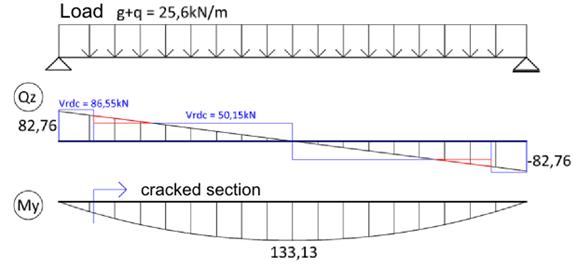$\textsf{\textit{\footnotesize{\qquad Shear resistance comparison before and after the cracks occurred.}}}$To the expression of VRd, c according to Article 6.2.2 (2) it must also be noted that in the general case should be based on check at the fiber of the extreme principal concrete tensile stress in the zone of normal compressive stress, but not at the center of gravity of the section. At this point it is necessary to calculate the cross-sectional characteristics (S and bW). To determine the maximum principal stress s1 in program IDEA RCS we draw a line through the centre of gravity in the direction of the resultant shear forces. This line we divide to 20 sectors. On this line we will present more characteristic points (points of the cross-section polygon, centre of gravity, the neutral axis). Within these points, we calculate S, bw, σx, τyz a σ1. At the point of maximum principal tensile stress we will calculate the shear resistance. Shear force before applying the reduction factor b required by Article 6.2.2 (6) must satisfy the extra condition${{V}_{Ed}}\le 0,5~{{b}_{w}}d~\upsilon ~{{f}_{cd}}$where ${{ υ}}\le 0,6\left[ 1-\frac{{{f}_{ck}}}{250} \right]$ kde fck je v MPaShear resistance of members without reinforcement or lightly reinforced (art. 12.6.3 )Shear resistance for plain or lightly reinforced concrete can be determined from the expression${{\tau }_{cp}}\le k~{{V}_{Ed~}}/{{A}_{cc}}$Whereτcp we substitute by${{f}_{cvd}}=\sqrt{f_{ctd,pl}^{2}+{{\sigma }_{cp}}{{f}_{ctd,pl}}}~pro~{{\sigma }_{cp}}\le {{\sigma }_{c,lim}}~$or${{f}_{cvd}}=\sqrt{f_{ctd,pl}^{2}+{{\sigma }_{cp}}{{f}_{ctd,pl}}-{{\left( \frac{{{\sigma }_{cp}}-{{\sigma }_{c,lim}}}{2} \right)}^{2}}}~pro~{{\sigma }_{cp}}>{{\sigma }_{c,lim}}~$Partial values used in the above formula are given by:${{\sigma }_{c,lim}}={{f}_{cd,pl}}-2\sqrt{{{f}_{ctd,pl}}\left( {{f}_{ctd,pl}}+{{f}_{cd,pl}} \right)}$where fcd,pl Design compressive strength for plain or lightly reinforced concrete,fctd,pl Design axial tensile strength of plain or lightly reinforced concrete,fcvd Design shear resistance under concrete compression.The resistance of members with shear reinforcement (art. 6.2.3 )Calculation of reinforced concrete members resistance with shear reinforcement is based on the truss analogy method with variable-angle diagonals. The basis of this method is the balance of forces in the triangle determined by the strut force (diagonal), the shear reinforcement force (stirrup) and longitudinal reinforcement force.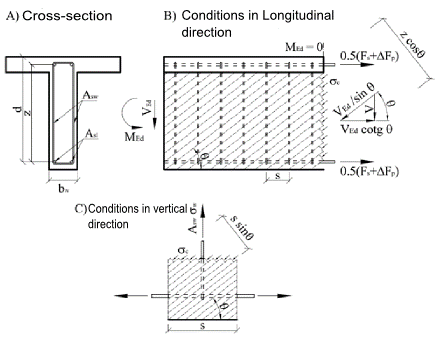$\textsf{\textit{\footnotesize{\qquad Principe of Truss analogy for member under shear load.}}}$Cross-section under shear load is broken by cracks at an angle θ, from this reason the concrete diagonal with same angle as shear forces is resisting to the shear force. Compressive force of the diagonal can be expressed as Ved/sinθ. This force must be transferred by concrete surface, perpendicular to the compression diagonal bwzcosθ. The concrete tension stress in the compression diagonal is then equal:${{\sigma }_{c}}=\frac{{{V}_{Ed}}}{{{b}_{w}}z~\sin \text{ }\!\!\theta\!\!\text{ }\cos \theta }=\frac{{{V}_{Ed}}}{{{b}_{w}}z}\left( \tan \theta +\cot \theta \right)$Substituting ${{\sigma }_{c}}={{\alpha }_{cw}}{{\nu }_{1}}{{f}_{cd}}$ and ${{V}_{Ed}}={{V}_{Rd,max}}$ and expressing ${{V}_{Rd,max}}$ we get equation for shear resistance of diagonal:${{V}_{Rd,max}}=~{{\alpha }_{cw}}~{{b}_{w}}~z~{{\nu }_{1~}}{{f}_{cd}}/\left( \cot \theta +\tan \theta \right)$To balance the vertical force component in the compression diagonal, shear reinforcement will be used. The size of the vertical force is based on the diagonal compressive stress in the concrete area which is corresponding to one single stirrup - ${{\sigma }_{c}}{{b}_{w}}s{{\sin }^{2}}\theta$. Limit stirrup force is given as ${{A}_{sw}}{{f}_{ywd}}/s$. Inserting σc, comparing with the limit force in the reinforcement, after modifications we get:$\frac{{{A}_{sw}}{{f}_{ywd}}}{s}=\frac{{{V}_{Ed}}}{z}\tan \theta$Then expressing Ved as VRDs we get resistance of cross-section with vertical shear reinforcement:${{V}_{Rd,s}}=~\frac{{{A}_{sw}}}{s}z~{{f}_{ywd}}\cot \theta$The longitudinal shear force is transferred by longitudinal reinforcement and it can be determined as Vedcotgθ . Derivation of formulas above can be found in .Using the program IDEA RCS it is possible to check only members with vertical shear reinforcement. In general following equations can be used:${{V}_{Rd,s}}=~\frac{{{A}_{sw}}}{s}z~{{f}_{ywd}}\left( \cot \theta +\cot \alpha \right)\sin \alpha$${{V}_{Rd,max}}=~{{\alpha }_{cw}}~{{b}_{w}}~z~{{\nu }_{1~}}{{f}_{cd}}\left( \cot \theta +\cot \alpha \right)/\left( 1+{{\cot }^{2}}\theta \right)$Where Asw is the cross-sectional area of the shear reinforcement,s is the spacing of the stirrups,fywd is the design yield strength of the shear reinforcement,bw is the minimum width between tension and compression chords. To calculate the resistance VRd,max , the value of the section width must be reduced to the so-called nominal width of the cross-section in case the cross-section is weakened by cable ducts bw,nom=bw-0,5ΣΦ for grouted metal ducts bw,nom=bw-1,2ΣΦ for non-grouted metal ducts υ = 0,6 pro fck ≤ 60MPa or pro fck > 60MPa,αcw is a coefficient taking account of the state of the stress in the compression chord. Loadσcp = 00 < σcp≤0,25 fcd0,25 fcd < σcp≤0,5 fcd0,5 fcd < σcp≤1,0 fcd Coefficient acw1,01+σcp/fcd1,252,5(1 - σcp/fcd) Tab. 1‑1 Determining coefficient αcwAngle θ is the angle between the concrete compression strut and the beam axis perpendicular to the shear force. The limiting values of cotθ for use in a Country may be found in its National Annex. The recommended limits are given by the expression:$1~\le ~\cot \theta \le 2,5$Choosing the size of the angle θ can affect the value of the resistances. Dependence of resistances is visible in Figure 1.15. The figure shows that with increasing of angle θ the resistance VRd,max is increasing, and resistance VRd,s is decreasing. Resistance VRd,c is constant, since it is based on the truss analogy method.$\textsf{\textit{\footnotesize{\qquad Dependency between shear resistance and angle q.}}}$Cross-section characteristics calculation for shearTo calculate the shear it is important to calculate the cross-sectional variables affecting the shear resistance. These variables include mainly shear-resisting section width bw, the effective depth d and lever arm z. The code  gives these values that directly correlate with the actual bending stress. But the problem is to determine these values when the direction of the resultant bending moments (or more accurately the direction of the resultant of section resistance) is significantly different from the direction of the resultant shear forces. In this case, the EC2 code doesn’t provide any recommendations.Cross-section width resisting to shear bwThe IDEA RCS program calculates the cross-section width resistant to shear in the direction perpendicular to the resultant of shear forces. Depending on the article in the Eurocode this width is calculated as: - The smallest width of the section between the resultant of compressive concrete and tensile reinforcement in the direction perpendicular to the resultant of shear forces for article 6.2.2 (a) and 6.2.3 (1) - The section width in a direction perpendicular to the resultant of shear forces in the checked point according to article 6.2.2 (2)Effective depth of a cross-sectionEffective depth is usually defined as the distance of most compressed concrete fiber to the centre of gravity of the reinforcement. Because it is directly related to the bending, the distance is given as perpendicular projection to the gravity line of the plane strain. This definition can be clarified so that instead of the centre of gravity of the tensile reinforcement the position of the reinforcement resultant of forces is used. During the development the IDEA RCS program the problem was solved: how to define the effective depth of the cross-section, for which the plane of bending loads doesn’t correspond with the direction of the resultant shear forces. Therefore, the effective depth is defined as the distance of most compressed concrete fibre to the resultant forces in the tensile reinforcement (based on bending stress) and in the direction of the resultant shear forces, see Figure 1.17.Exceptional cases will occur if we are not able to determine the compressed fiber or resultant in the tensile reinforcement. In this case, we recommend using value 0.9 h (90% of section depth in the direction of the resultant shear forces). This value, the user can define in the IDEA RCS program by the setting of code variables.Lever arm of internal forcesThe lever arm of internal forces is in 6.2.3 (3)  and is defined as the "distance between tension and compression chords”. The code does not define how to proceed when the plane of acting bending moment is different from the direction of resultant shear forces. Therefore, as for the case of the effective depth, we define the distance in the direction of the resultant shear forces. Also here, we can face a similar exception cases, for example, the whole section is under compression, etc. In this case, we take value 0.9 d (90% of the effective section height). This value, the user can set in the IDEA RCS program by the setting of code variables.Dependence between bending plane inclination and the resultant of shear force is clearly visible in Figure 1.18 and Figure 1.19. With an increase of inclination the values of effective height, lever arms and related resistances are decreasing. The limit state is 90°. For this inclination the lever arm of internal forces cannot be calculated, consequently the lever arm is equal to zero. In this case, the value specified in the setting of code variables is considered. By this, there is a jump at the end of the chart. This study proves the recommended maximum for inclination is about 20°.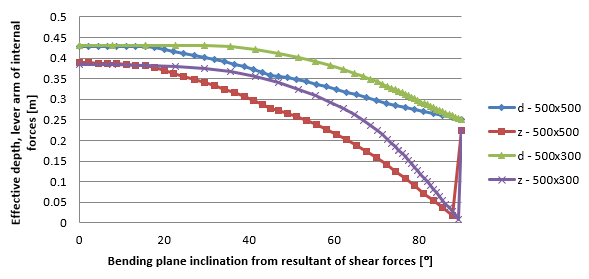$\textsf{\textit{\footnotesize{\qquad Dependence between effective depth, lever arm to the bending plane inclination and the resultant of shear forces.}}}$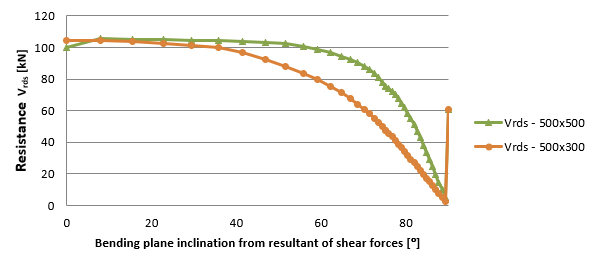$\textsf{\textit{\footnotesize{\qquad Dependence between resistance Vrds to the bending plane inclination and the resultant of shear.}}}$As part of the testing of the RCS application, a study about dependency of shear resistance to changing the normal force was conducted. Resistance VRd,max is affected only by the coefficient αcw, see Fig. 1.20. Fig. 1.21 shows a constant value of resistance VRds. For VRdc resistance, the decreases cause increasing of normal force. The blue curve in Fig. 1.21 shows the resistance VRdc with neglecting the influence of cracks and it was calculated using the formula in section 6.2.2 (1) . Jump in transition between pressure and tension is caused by contributed tensile reinforcement. The red curve is calculated using the formula in section 6.2.2 (2) . After the first crack occurred the dependency curve is same as for 6.2.2 (1) .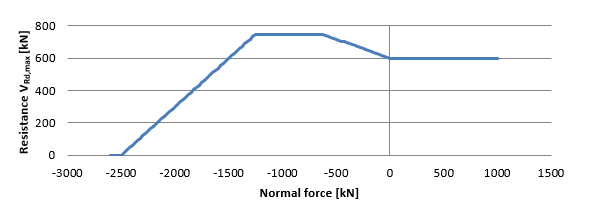$\textsf{\textit{\footnotesize{\qquad Dependency curve of shear resistance VRd,max to normal force.}}}$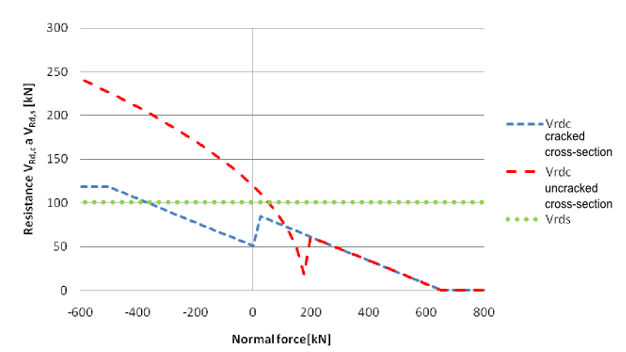$\textsf{\textit{\footnotesize{\qquad Dependency of shear resistances VRd,c a VRd,s to normal force.}}}$ Torsion Calculation assumptionsThe behavior of a reinforced concrete section subjected to torsion can be divided into two categories - before and after the time when the cracks may first be expected to occur. Before a crack the cross-section behaves as an elastic material. Torsion stress can be expressed by formula $\tau =~\frac{{{T}_{Ed}}}{{{W}_{t}}}$ where Wt je sectional module in torsion.Cracks in the unreinforced member due to principal tensile torsion stress are also ultimate limit state. The behavior of a reinforced concrete section subjected to torsion can be described on the basis of a thin-walled closed section, see Fig. below.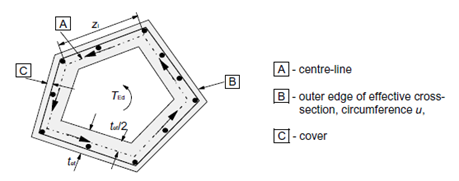$\textsf{\textit{\footnotesize{\qquad Equivalent thin-walled cross-section.}}}$Calculation procedureThe process of a reinforced concrete check for torsion is very similar to the check for shear. First of all, we check the concrete resistance. If the concrete check is satisfied, the reinforcement can be designed using the detailing rules. Otherwise, we need to verify the reinforcement and compressive diagonal resistance by calculation.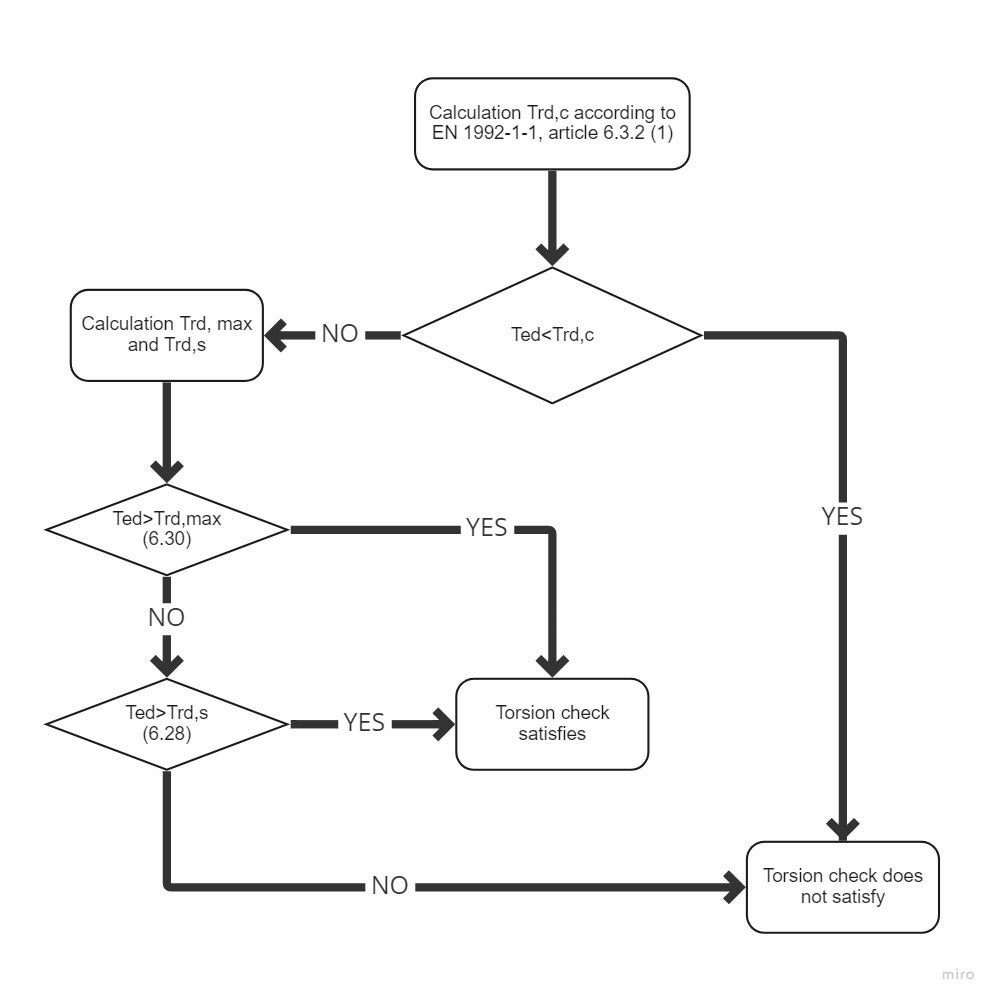$\textsf{\textit{\footnotesize{\qquad Process diagram for torsion check.}}}$ResistanceShear flow in a wall of a thin-walled cross-section under torsion can be expressed as:${{\tau }_{t}}{{t}_{ef}}=~\frac{{{T}_{Ed}}}{2{{A}_{k}}}$Shear force in a wall of a thin-walled cross-section can be expressed as:$V={{\tau }_{t}}{{t}_{ef}}z$Where τ Shear flow in wall,tef is the effective wall thickness,z is the side length of wall,TEd is the torsion moment,Ak is the area enclosed by the center-lines of the connecting walls, including inner hollow areas.Torsion cracking moment, which may be determined by setting fctd to previous expression. Thus we get expression for the resistance in torsion without torsion reinforcement.${{T}_{Rd,c}}=2{{A}_{k}}{{t}_{ef}}{{f}_{ctd}}$where fctd design axial tensile strength of concrete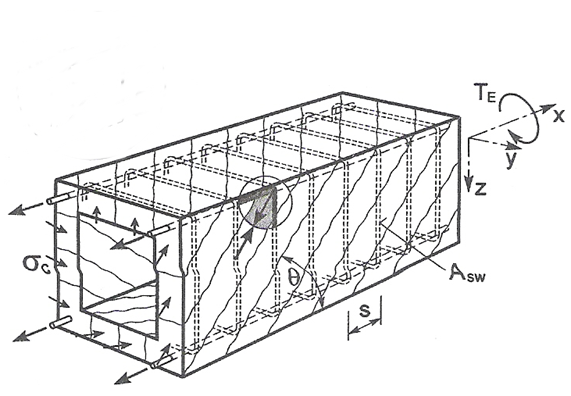$\textsf{\textit{\footnotesize{\qquad Principles of Truss analogy for member under torsion moment.}}}$The member resistance with torsion reinforcement is composed from the resistance of compressive concrete diagonals which is based again on the truss analogy method. Compressive stress in the diagonal can be expressed with help of shear force in the wall of a thin-walled cross-section on the wall surface which is in consideration, i.e.${{\sigma }_{c}}=\frac{\frac{{{T}_{Ed}}z}{2{{A}_{k}}\sin \theta }}{z~{{t}_{ef}}\cos \theta }=\frac{{{T}_{Ed}}}{2{{A}_{k}}{{t}_{ef}}\sin \theta \cos \theta }$Substitution of σc=σcwfcd and TEd=TRd,max and expressing of TRd,max we get an equation for the compressive diagonal resistance${{T}_{Rd,max}}=2~\nu ~{{\alpha }_{cw}}~{{f}_{cd}}~{{A}_{k}}~{{t}_{ef~\sin \theta ~\cos \theta }}$where ν = 0,6 pro fck ≤ 60MPa or for fck > 60MPaαcw coefficient which takes account the state of compressive stress in compression chordfcd design value of concrete compressive strengththe shear reinforcement resistance subjected to torsion is again based on stress in the compression diagonal. The stirrup force is equal to the stress in the compressed diagonal on the area which corresponds to the particular stirrup line, i.e.${{A}_{sw}}{{f}_{ywd}}=\frac{{{T}_{Ed}}}{2{{A}_{k}}{{t}_{ef}}\sin \theta \cos \theta }~{{t}_{ef}}~s{{\sin }^{2}}\theta =\frac{{{T}_{Ed}}~s}{2{{A}_{k}}\cot \theta }~$Substituting TEd=TRd,s and expressing TRd,s we get equation: ${{T}_{Rd,s}}=2{{A}_{k}}\frac{{{A}_{sw}}{{f}_{ywd}}}{s}~\cot \theta$ If the longitudinal and shear reinforcement quantity is known, we can define angle θ by the expression${{\tan }^{2}}\theta =\frac{\frac{{{A}_{sw}}{{f}_{ywd}}}{s}}{\frac{{{A}_{sl}}{{f}_{yd}}}{{{u}_{k}}}}$ Substitution for TRd,s we get${{T}_{Rd,s}}=2{{A}_{k}}\sqrt{\frac{{{A}_{sw}}}{s}{{f}_{ywd~}}\frac{{{A}_{sl}}}{{{u}_{k}}}~{{f}_{yd}}}$ WhereAsw shear reinforcement areas is the radial spacing of stirrups of shear reinforcementfywd is the effective design strength of the shear reinforcementAsl longitudinal reinforcement areauk is the outer circumference of the cross-sectionfywd is the effective design strength of the longitudinal reinforcementThe force in the longitudinal reinforcement can be deducted from The shear force in a wall of a section subject to a pure torsional moment, which is give as:$V=\frac{{{T}_{Ed}}}{2{{A}_{k}}}{{u}_{k}}$That force is transformed to longitudinal direction and we get:${{F}_{l}}=\frac{{{T}_{Ed}}{{u}_{k}}}{2{{A}_{k}}~\tan \theta }$The permitted range of the values for angle θ is similar to the shear check, i.e 1 < cot θ < 2,5. Dependency between resistances can be seen in Fig. below. The diagram shows that with increasing the angle θ the resistance TRd,max is growing, the resistance TRd.s is decreasing and resistance TRd,c is constant, since it is not based on the truss analogy method.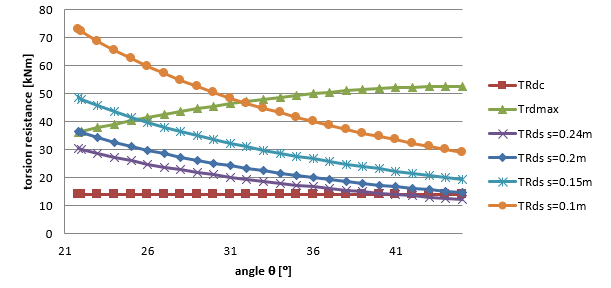$\textsf{\textit{\footnotesize{\qquad Závislost únosnosti průřezu v kroucení na úhlu θ.}}}$Calculation of cross-section characteristics for torsionTo check the cross-section for torsion it is necessary to establish a so-called equivalent thin-walled closed section. In determining the dimensions of the equivalent thin-walled cross-section by assuming a rectangular shape. For the true area of rectangular states A = b×h and for the circumference of rectangle u =2 (b +h). Using these two equations can provide alternative thin rectangle-shaped area and periphery of the original cross-section. Solving two equations with two unknowns we get:$b=\frac{-u\pm \sqrt{{{u}^{2}}-16A}}{-4}\text{ }\!\!~\!\!\text{ }$$h=\frac{\left( u-2\text{b} \right)}{2}$The wall thickness of the effective cross-section can be define from periphery and section area as:$t=\text{A}/\text{u}$Then the area and periphery defined by center line of the effective cross-section:${{A}_{k}}=\left( \text{h}-\text{t} \right)\text{ }\!\!~\!\!\text{ }\left( \text{b}-\text{t} \right)\text{ }\!\!~\!\!\text{ }$${{u}_{k}}=2\left( \left( \text{h}-\text{t} \right)+\text{ }\!\!~\!\!\text{ }\left( \text{b}-\text{t} \right) \right)$The problem with this method is for cross-section of type T with a wide plate when the overall area and periphery is taken to calculate the dimensions (including this plate). In the future versions of IDEA RCS program, the selection of the most massive cross-section part will be enabled, which will be used to check the torsion. Interaction Interaction of shear force and torsion for shear reinforcementDetermination of the force in shear reinforcement due to shear force.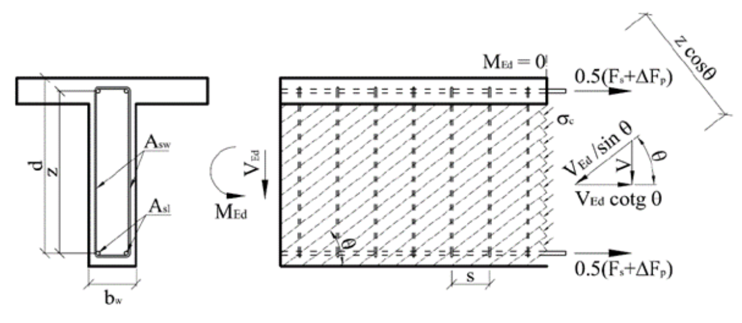The calculation is based on the formula for calculating the resistance of the shear reinforcement defined in EN 1992-1-1. Based on equation 6.13 (chap. 6.2.3 (4)) the load-bearing resistance of one stirrup leg can be derived as:${{V}_{Rd,s}}=\frac{{{A}_{sw,V}}}{s}z{{f}_{ywd}}\left( \cot \theta +\cot \alpha \right)\sin \alpha \cos \beta$$\frac{{{A}_{sw,V}}}{s}={{a}_{sw,V}}$Asw,V . . . cross-sectional area of one stirrup leg resisting shear in considered sections . . . . . spacing of the shear reinforcement in the direction of the longitudinal member axis asw,V . . . cross-sectional area of the shear reinforcement per unit lengthz . . . . . the inner lever arm. For a member with constant depth, corresponding to the bending moment in the element under consideration. In the shear analysis of reinforced concrete without axial force, the approximate value z = 0,9d may normally be used.fywd . . . the design yield strength of shear reinforcementθ . . . . . the angle between the concrete compression strut and the member axis perpendicular to the shear forceα . . . . . the angle between shear reinforcement and the member axis perpendicular to the shear forceβ . . . . . inclination of the leg of the stirrup relative to the resultant of the applied shearing force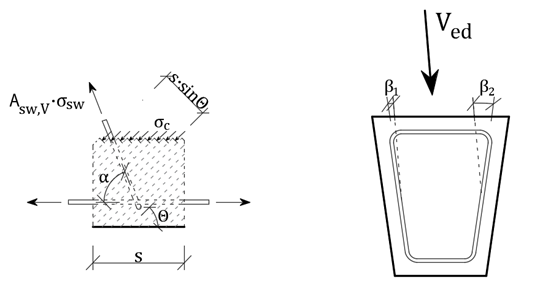The shear force is redistributed evenly among the individual reinforcement resisting the shear force based on the angle of the reinforcement and the axial stiffness of the individual stirrup legs.${{V}_{ed}}={{V}_{ed,1}}+{{V}_{ed,2}}+...+{{V}_{ed,n}}$${{V}_{ed}}={{\varepsilon }_{sw,V}}\cdot z\cdot \sum\limits_{i=1}^{{{n}_{V}}}{{{a}_{sw,i,V}}\cdot {{E}_{sw,i,V}}\cdot \left( \cot \theta +\cot {{\alpha }_{i}} \right)\cdot {{\cos }^{2}}{{\beta }_{i}}}$Further, the average reinforcement strain considered in the direction of the resultant shear force can be derived:${{\varepsilon }_{sw,V}}=\frac{{{V}_{ed}}}{z\cdot \sum\limits_{i=1}^{{{n}_{V}}}{{{a}_{sw,i,V}}\cdot {{E}_{sw,i,V}}\cdot \left( \cot \theta +\cot {{\alpha }_{i}} \right)\cdot {{\cos }^{2}}{{\beta }_{i}}}}$The actual strain of the i-th reinforcement can be calculated as:${{\varepsilon }_{sw,i,V}}=\frac{{{\varepsilon }_{sw,V}}}{\sin {{\alpha }_{i}}}\cdot \cos {{\beta }_{i}}$The tension in a given leg of the reinforcement:${{\sigma }_{sw,i,V}}={{\varepsilon }_{sw,i,V}}\cdot {{E}_{si,V}}$Determination of the force in individual stirrup due to torsionThe torsional resistance of a section may be calculated on the basis of a thin-walled closed section, in which equilibrium is satisfied by a closed shear flow. Solid sections may be modeled by equivalent thin-walled sections. For non-solid sections, the equivalent wall thickness should not exceed the actual wall thickness. Shear flow in the walls of a thin-walled closed section due to torsion may be calculated as:${{\tau }_{t}}\cdot {{t}_{ef}}=\frac{{{T}_{ed}}}{2{{A}_{k}}}$Shear force in a particular wall is then:${{V}_{i}}={{\tau }_{t}}\cdot {{t}_{ef}}\cdot {{l}_{i}}$li . . . . length of the centreline of the wall under considerationShear force in the web - the length of the web centreline can be substituted by the value of the lever arm "z".${{V}_{ed,T}}=\frac{{{T}_{ed}}}{2{{A}_{k}}}\cdot z$Force in stirrups resisting torsion per one meter of the member length (per unit length):${{F}_{sw,T}}=\frac{{{V}_{ed,T}}}{z\cdot \cot \theta }=\frac{{{T}_{ed}}}{2{{A}_{k}}}\cdot tg\theta$Decompositions of forces for individual stirrupIf the same material is defined for all stirrups, the resulting stress due to torsion in each stirrup leg is constant. Then:${{\sigma }_{sw,T}}=\frac{{{F}_{sw,T}}}{{{a}_{sw,T}}}$where asw,T is the total area of stirrups resisting the torsion per unit length.In the case that individual stirrups have different materials, the axial stiffness of the individual bars must be taken into account.${{F}_{sw,T}}={{F}_{s1,T}}+{{F}_{s2,T}}+{{F}_{s3,T}}+...+{{F}_{sn,T}}=\sum\limits_{i=1}^{{{n}_{T}}}{{{F}_{si,T}}}$${{\varepsilon }_{sw,T}}=\frac{{{F}_{sw,T}}}{\sum\limits_{i=1}^{{{n}_{T}}}{\left( {{a}_{si,T}}\cdot {{E}_{si,T}} \right)}}$nT . . . . number of legs of reinforcement (groups of reinforcement) resisting torsionFsi,T . . . force in the i-th group of reinforcement resulting from torsion per unit lengthasi,T . . . cross-sectional area of the shear reinforcement resisting torsion per unit length Esi,T . . . Young´s modulus of elasticity of the i-th group of reinforcement resisting torsionεsw,T . . strain in the reinforcement due to torsion The resulting stress in each stirrup due to applied torsion is calculated as:${{\sigma }_{sw,i,T}}={{\varepsilon }_{sw,T}}\cdot {{E}_{si,T}}$V+T interactionThe calculation of the stresses in stirrups due to shear and torsion is then a summation of the stresses due to individual load components. ${{\sigma }_{sw,i}}={{\sigma }_{sw,i,V}}+{{\sigma }_{sw,i,T}}$Resulting force in the i-th reinforcement:${{F}_{sw,i}}={{a}_{sw,i}}\cdot {{\sigma }_{sw,i}}$Interaction of shear, torsion and bending for longitudinal reinforcementDetermination of the force in each longitudinal reinforcement due to the normal force and bending momentThe application RCS is used to calculate the cross-sectional response due to the combination of the normal force and bending moment to determine the stress and strain in the individual longitudinal bars and prestressing reinforcement.Determination of the force in the individual longitudinal reinforcement due to shear forceThe increment of the tensile force in the longitudinal reinforcement ΔFtd from the shear force depends on the geometry of the strut and tie model. $\Delta {{F}_{td}}={{V}_{ed}}\left( \cot \theta -\cot \alpha \right)$ΔFtd . . . increment of tensile force in the longitudinal reinforcement due to the shear forceVed . . . . design value of the shear force acting in the section under considerationθ . . . . . the angle between the concrete compression strut and the member axis α . . . . . the angle between shear reinforcement and the member axisFor the longitudinal reinforcement located in the tension chord, the resulting force Ft in the longitudinal reinforcement due to the combination N+M+V should be taken not greater than MEd,max/z (where MEd,max is the maximum moment along the beam)${{F}_{t}}=\frac{{{M}_{Ed}}}{z}+0,5{{V}_{ed}}\left( \cot \theta -\cot \alpha \right)\le \frac{{{M}_{Ed,\max }}}{z}$The force ΔFtd is transmitted by all bonded prestressing tendons and reinforcement located in the part of the cross-section that resists shear (the web in the case of an I-profile). On the safe side, the contribution of prestressing reinforcement can be considered 0. The assumption of the calculation is that the increment of the axial strain of the individual longitudinal reinforcement resisting shear is constant (Δεs1,V = Δεs2,V = .... =Δεp1,V = Δεp2,V = ... = ΔεV = const.). The derivation is valid for a bilinear reinforcement working diagram with a horizontal plastic branch. In the case of a diagram with an inclined branch, the calculation must be modified.$\Delta {{F}_{td}}=\Delta {{F}_{s}}+\Delta {{F}_{s}}$$\Delta {{F}_{td}}=\Delta {{\varepsilon }_{V}}\cdot \sum\limits_{i=1}^{{{n}_{s,V}}}{{{A}_{sl,i,V}}\cdot {{E}_{sl,i,V}}}+\Delta {{\varepsilon }_{V}}\cdot \sum\limits_{i=1}^{{{n}_{p,V}}}{{{A}_{pl,i,V}}\cdot {{E}_{pl,i,V}}}$ΔεV . . . . strain increment in the longitudinal reinforcement due to the shear forcens,V . . . . number of longitudinal reinforcements resisting the shear forceAsl,i,V . . . area of the i-th longitudinal reinforcement resisting the shear forceEsl,i,V . . . Young´s modulus of elasticity of the i-th longitudinal reinforcement resisting the shear forcenp,V . . . . number of tendons resisting the shear forceApl,i,V . . . area of the i-th tendon resisting the shear forceEpl,i,V . . . Young´s modulus of elasticity of the i-th tendon resisting the shear forceAfter determining the value of force ΔFtd, the average reinforcement strain ΔεV can then be calculated.$\Delta {{\varepsilon }_{V}}=\frac{\Delta {{F}_{td}}}{\sum\limits_{i=1}^{{{n}_{s,V}}}{{{A}_{sl,i,V}}\cdot {{E}_{sl,i,V}}}+\sum\limits_{i=1}^{{{n}_{p,V}}}{{{A}_{pl,i,V}}\cdot {{E}_{pl,i,V}}}}$Stress increment in the individual longitudinal bars due to the applied shear force:for rebar $\Delta {{\sigma }_{sl,i,V}}=\Delta {{\varepsilon }_{V}}\cdot {{E}_{sl,i,V}}$for tendon $\Delta {{\sigma }_{pl,i,V}}=\Delta {{\varepsilon }_{V}}\cdot {{E}_{pl,i,V}}$Determination of the force in each longitudinal reinforcement from torsionIt is very important to determine the longitudinal reinforcement that resist the torsion. These are the reinforcement that are located in a torsion-resisting alternate effective thin-walled cross-section.$\frac{\sum{{{A}_{sl}}{{f}_{yd}}}}{{{u}_{k}}}=\frac{{{T}_{ed}}}{2{{A}_{k}}}\cot \theta$According to EN 1992-1-1, several conditions must be fulfilled for longitudinal torsion resistant reinforcement:- the reinforcement should be uniformly distributed along the length zi, but in small cross-sections the reinforcement may be concentrated at the corners of the stirrup- the maximum axial distance of the longitudinal reinforcement is 350 mmThe contribution of prestressing reinforcement is not considered according to EN 1992-1-1.The code EN 1992-2 states that the contribution of prestressing reinforcement may be considered, but the maximum stress increment in the prestressing reinforcement shall not exceed Δσp ≤ 500MPa. Then the formula can be modified:$\frac{\sum{{{A}_{sl}}{{f}_{yd}}+\sum{{{A}_{p}}\Delta {{\sigma }_{p}}}}}{{{u}_{k}}}=\frac{{{T}_{ed}}}{2{{A}_{k}}}\cot \theta$However, since the increment of prestressing reinforcement can be considered, but it is at the user's choice. Currently, prestressing reinforcement is not considered in the calculation. The assumption of the calculation is that the increment of the axial strain of each longitudinal shear resisting reinforcement is constant (Δεs1,T = Δεs2,T = .... =Δεp1,T = Δεp2,T = ... = ΔεT = const.). The derivation is valid for a bilinear reinforcement working diagram with a horizontal plastic branch. In the case of a diagram with an increasing branch, the calculation must be modified.$\frac{\sum\limits_{i=1}^{{{n}_{T}}}{{{A}_{sl,i,T}}\cdot \Delta {{\sigma }_{s,i,T}}}}{{{u}_{k}}}=\frac{{{T}_{ed}}}{2{{A}_{k}}}\cot \theta$$\frac{\sum\limits_{i=1}^{{{n}_{T}}}{{{A}_{sl,i,T}}\cdot \Delta {{\varepsilon }_{T}}\cdot {{E}_{s,i,T}}}}{{{u}_{k}}}=\frac{{{T}_{ed}}}{2{{A}_{k}}}\cot \theta$$\Delta {{\varepsilon }_{T}}=\frac{{{T}_{ed}}\cdot {{u}_{k}}}{2{{A}_{k}}\cdot \sum\limits_{i=1}^{{{n}_{T}}}{{{A}_{sl,i,T}}\cdot {{E}_{s,i,T}}}}\cot \theta$$\frac{\sum\limits_{i=1}^{{{n}_{T}}}{{{A}_{sl,i,T}}\cdot \Delta {{\sigma }_{s,i,T}}}}{{{u}_{k}}}=\frac{{{T}_{ed}}}{2{{A}_{k}}}\cot \theta$$\frac{\sum\limits_{i=1}^{{{n}_{T}}}{{{A}_{sl,i,T}}\cdot \Delta {{\varepsilon }_{T}}\cdot {{E}_{s,i,T}}}}{{{u}_{k}}}=\frac{{{T}_{ed}}}{2{{A}_{k}}}\cot \theta$$\Delta {{\varepsilon }_{T}}=\frac{{{T}_{ed}}\cdot {{u}_{k}}}{2{{A}_{k}}\cdot \sum\limits_{i=1}^{{{n}_{T}}}{{{A}_{sl,i,T}}\cdot {{E}_{s,i,T}}}}\cot \theta$Ted . . . . the design value of the torque applied in the section under considerationθ . . . . . inclination of the compression diagonals with respect to the longitudinal axis of the beam (identical to that for the shear force)uk . . . . perimeter of the area AkAf . . . . the area defined by the centreline of the replacement hollow thin-walled sectionns,T . . . .number of longitudinal concrete reinforcement resisting torqueAsl,i,T . . . area of the i-th longitudinal concrete reinforcement resisting torqueΔεT . . . .the change in the longitudinal reinforcement transformation due to the torqueΔσs,i,T . . change in stress in the i-th longitudinal reinforcement due to torqueEsl,i,T . . . modulus of elasticity of the i-th longitudinal concrete reinforcement resisting the torqueStress increment in each longitudinal reinforcement from the applied torque:$\Delta {{\sigma }_{sl,i,T}}=\Delta {{\varepsilon }_{T}}\cdot {{E}_{sl,i,T}}$ Stress limitation check The check is based on general assumptions, where two states of the cross-section are resolved: the uncracked section (the tensile strength of the concrete is not ignored) and the fully cracked section (the tensile strength of the concrete is ignored). The solution with ignored concrete tensile strength is considered under the assumptions of Article 7.1 (2) EN 1992-1-1.When calculating the stress and deflections it is considered as an uncracked section, if the tensile stress in bending does not exceed fct, eff. The value of fct, eff can be considered as fctm or fctm,fl. The fctm value is used when calculating the crack width and tensile stiffening.As part of this check, we deal with four basic cases in terms of stress limit. 7.2 (2) Compressive stress in members exposed to environments of exposure classes XD, XF and XS should be limited: $\left| {{s}_{c}} \right|\le {{k}_{1}}{{f}_{ck}}$${{k}_{1}}=0,6$ 7.2 (3) The stress in the concrete under the quasi-permanent loads is limited: $\left| {{s}_{c}} \right|\le {{k}_{2}}{{f}_{ck}}$${{k}_{2}}=0,45$ 7.2 (5) Tensile stresses in the reinforcement under the characteristic combination of loads shall be limited: $\left| {{s}_{s}} \right|\le {{k}_{3}}{{f}_{yk}}$${{k}_{3}}=0,8$ 7.2 (5) Where the stress is caused by an imposed deformation, the tensile stress should not exceed: $\left| {{s}_{s}} \right|\le {{k}_{4}}{{f}_{yk}}$${{k}_{4}}=1$Where values k1, k2, k3, k4 for use in a Country may be found in its National Annex. The recommended values are 0,8; 1 and 0,75 respectively, characteristic yield stress of the reinforcement, fck characteristic cylinder strength fck determined at 28 days. Cracks The formation of cracksA characteristic feature of reinforced concrete structures under bending or tensile stress is the occurrence of cracking failure at points where the tensile stress in the concrete exceeds the tensile strength of the concrete. For the durability of the structure and also for the aesthetics of the structure, it is important to ensure that the resulting cracks are as small as possible. The calculation of the crack widths as well as the maximum widths allowed for the different exposure classes are given in EN 1992-1-1, Chapter 7.3.In the first step of the calculation, it is determined whether the cross-section is cracked or not. The crack width itself is always calculated from the quasi-permanent or frequent load combination (depending on the national annex), but the crack formation has to be checked from all specified SLS combinations. Thus, two cases can occur: The maximum tensile stress in the concrete fibers will not exceed the tensile strength of the concrete from any load combination (quasi-permanent ME,qp, frequent ME,fr, or characteristic ME,k), and hence we consider the cross-section without cracks. ${{M}_{E,i}}\le {{M}_{cr}}={{f}_{ct,ef}}\frac{{I}_{I}}{h-{{a}_{I}}}$ If cracks develop for any of the combinations (quasi-permanent, frequent, or characteristic), i.e. the bending moment developed from the considered load combination is greater than the critical moment Mcr, the cross-section is cracked from that load combination, and the characteristics of the cracked cross-section and the crack width have to be calculated. ${{M}_{E,i}}>{{M}_{cr}}={{f}_{ct,ef}}\frac{{I}_{I}}{h-{{a}_{I}}}$ME,i . . the bending moment obtained from some SLS load comb. Thus, it can be ME,qp, ME,fr, or ME,k. fct,ef . . the tensile strength of the concrete at the considered time. If the concrete is older than 28 days, we consider a strength equal to fctm.Crack width calculationIn a bending-loaded element, the crack formation is divided into 2 phenomena: Crack formation phase (stage number 2 in Fig. 1) Stabilized crack development (stage number 3 in Fig. 1)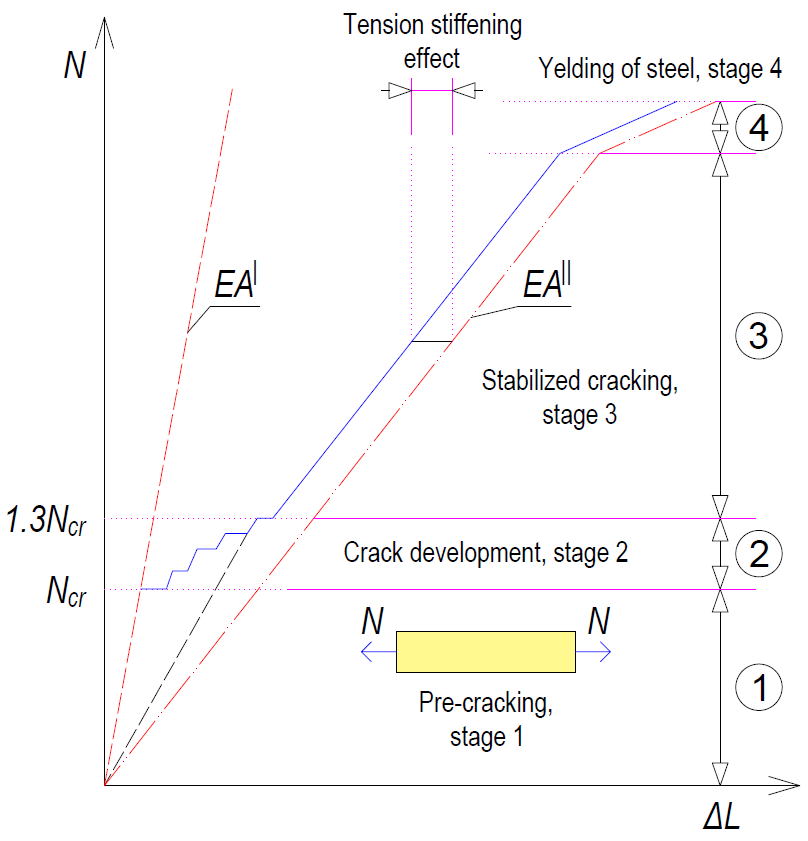$\textsf{\textit{\footnotesize{Fig. 1 Stages of the behavior of the reinforced concrete cross-section during loading}}}$Crack development stageThis is the initial part of the process when individual cracks are still gradually appearing until the entire tensile part of the member is affected by cracks that are approximately equally distributed along the length of the member. The first crack is formed when the force in the tensioned strip exceeds the value of the critical force Nr (Critical tensile force, see below), and further cracks develop up to a level of load exerting a force in the tensioned strip equal to approximately 1.3Ncr (phase number 2 in Fig. 1).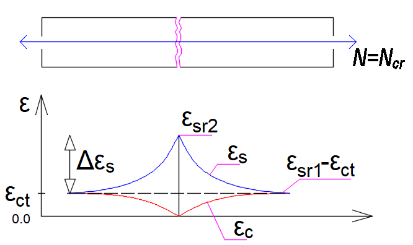$\textsf{\textit{\footnotesize{Fig. 2 Strains of concrete and reinforcement at the moment of the first crack}}}$The developing cracks are divided into 2 types - primary and secondary cracks. Primary cracks occur in the tensile fibers when the effective tensile strength of the concrete (fct,eff) is reached. Primary cracks represent the first pattern of cracks (Fig. 2). Shorter secondary cracks are then formed between the primary cracks (Fig. 3). At stresses corresponding to about 1.2 to 1.5 σsr (usually a mean value of 1.3 σsr is considered, where σsr is the stress in the reinforcement at the formation of primary cracks in the tensile zone of the concrete), the development of secondary cracks is also completed.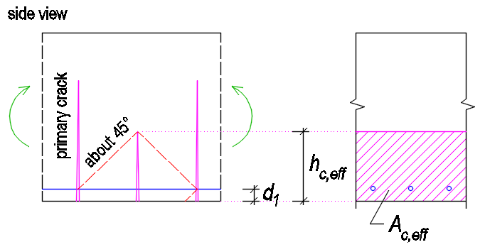$\textsf{\textit{\footnotesize{Fig. 3 Primary and secondary cracks}}}$The crack width at the crack formation stage can be calculated as follows:${{w}_{k}}=2{{l}_{s,\max }}\left( {{\varepsilon }_{sm}}-{{\varepsilon }_{cm}} \right)$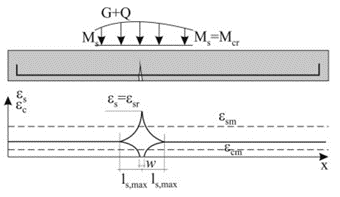$\textsf{\textit{\footnotesize{Fig. 4 Characteristics of the transmission length for the first crack}}}$Stabilized cracking stageAfter exceeding approximately 1.3 times the critical force in the tensile zone, no new cracks are formed, the number of cracks in the element is stabilized, and only the width of the existing cracks increase with further loading (stage number 3 in Fig. 1).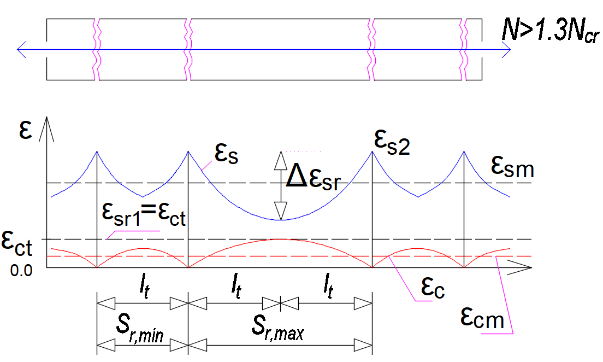$\textsf{\textit{\footnotesize{Fig. 5 Strains of concrete and reinforcement at the stabilized cracking stage}}}$The crack width during stable development can be calculated as:${{w}_{k}}={{s}_{r,\max }}\left( {{\varepsilon }_{sm}}-{{\varepsilon }_{cm}} \right)$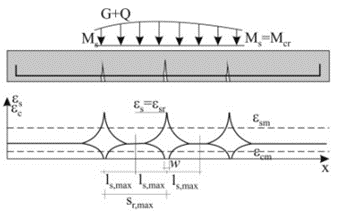$\textsf{\textit{\footnotesize{Fig. 6 Stabilized cracking}}}$Critical tensile forceThe calculation is based on the Tension Chord Model (TCM). The basic consideration is to calculate the ultimate capacity of a reinforced concrete strip formed by a reinforcing bar of area As,eff surrounded by an effective area of tensile concrete Ac,eff, which is able to resist the tensile stress until the tensile strength fct,eff is exceeded (normally we consider fctm). Assuming a perfect bond between the reinforcement and the concrete, we can consider that until the first crack occurs, the remodeling of the reinforcement and the surrounding concrete is identical. Then the maximum force in the tensile strip just before the first crack Nr can be determined:${{N}_{r}}={{A}_{c,eff}}\cdot {{f}_{ctm}}+{{A}_{s,eff}}\cdot {{\sigma }_{s}}$By introducing the substitution${{\alpha }_{e}}={}^{{{E}_{s}}}/{}_{{{E}_{cm}}};{{\rho }_{p,eff}}={}^{{{A}_{s,eff}}}/{}_{{{A}_{c,eff}}}$we get:${{N}_{r}}={{A}_{c,eff}}\cdot {{f}_{ctm}}\cdot \left( 1+{{\alpha }_{e}}\cdot {{\rho }_{p,eff}} \right)$Just after the formation of the first crack, the entire force Nr is transferred by the reinforcement and thus the stress in the reinforcement passing through the just-formed crack can be calculated as:${{\sigma }_{sr}}=\frac{{{f}_{ctm}}}{{{\rho }_{p,eff}}}\cdot \left( 1+{{\alpha }_{e}}\cdot {{\rho }_{p,eff}} \right)\Rightarrow {{\varepsilon }_{sr}}=\frac{{{f}_{ctm}}}{{{E}_{s}}\cdot {{\rho }_{p,eff}}}\cdot \left( 1+{{\alpha }_{e}}\cdot {{\rho }_{p,eff}} \right)$Crack width calculation according to EC 1992-1-1The following equation is used to calculate the width of cracks on reinforced concrete elements:${{w}_{k}}={{s}_{r,\max }}\left( {{\varepsilon }_{sm}}-{{\varepsilon }_{cm}} \right)$sr,max . . . maximum crack spacingεsm . . . . the average strain of the reinforcement from the load combination, including the effects of tension stiffening.εcm . . . . average strain of concrete between cracksCalculation of the strain differenceThe difference in the strain of reinforcement and concrete between cracks can be obtained from the equation:${{\varepsilon }_{sm}}-{{\varepsilon }_{cm}}=\frac{{{\sigma }_{s}}-{{k}_{t}}\cdot \frac{{{f}_{ct,eff}}}{{{\rho }_{p,eff}}}\cdot \left( 1+{{\alpha }_{e}}\cdot {{\rho }_{p,eff}} \right)\,}{{{E}_{s}}}\ge 0,6\frac{{{\sigma }_{s}}}{{{E}_{s}}}$σs . . . . the stress in the reinforcement in the crack from the load combination under considerationkt . . . . an empirical coefficient taking into account the average strain, depended on the duration of the load. It can take values of 0.6 for short-term analysis. For the long-term analysis, the reduction of the stiffness of the composite to about 70% is taken into account, so its value is 0.4, which includes the rate of degradation of the cohesion between the reinforcement and the concrete due to time.αe . . . . the effective ratio of elastic moduli${{\alpha }_{e}}={}^{{{E}_{s}}}/{}_{{{E}_{cm}}}$ςp,eff . . . . effective level of reinforcement${{\rho }_{p,eff}}={}^{\left( {{A}_{s,eff}}+{{\xi }^{2}_{1}}A_{p}^{\acute{\ }} \right)}/{}_{{{A}_{c,eff}}}$Ac,eff . . . . the effective area of the concrete in tension surrounding the reinforcement (determination of Ac,eff below)As,eff . . . . the area of bonded reinforcement located in the area of Ac,effAp´ . . . . is the area of pre- or post-tensioned tendons within Ac,effξ1 . . . . . is the adjusted ratio of bond strength, taking into account the different diameters of prestressing and reinforcing steel:${{\xi }_{1}}=\sqrt{\xi \,\cdot \,\frac{{{\phi }_{s}}}{{{\phi }_{p}}}}$ξ . . . the ratio of bond strength of prestressing and reinforcing steel (Table 6.2)ϕs . . largest bar diameter of reinforcing steelϕp . . the diameter or equivalent diameter of prestressing steelFor bundles, Ap is the area of reinforcement in the tendon${{\phi }_{p}}=1,6\sqrt{{{A}_{p}}}$For single seven-wire strands where φwire is the wire diameter${{\phi }_{p}}=1,75\,\,{{\phi }_{wire}}$For single three-wire strands where φwire is the wire diameter${{\phi }_{p}}=1,20\,\,{{\phi }_{wire}}$If only prestressing reinforcement is used to prevent cracking, then the following must be considered.${{\xi }_{1}}=\sqrt{\xi \,}$In prestressed members, a minimum area of bonded reinforcement is not required as long as, under the characteristic combination of loading and the characteristic value of prestressing force the tensile stress in any fiber is not greater than the tensile strength of the concrete, fct,eff. (see EN 1992-1-1 ch. 7.3.2 for more details)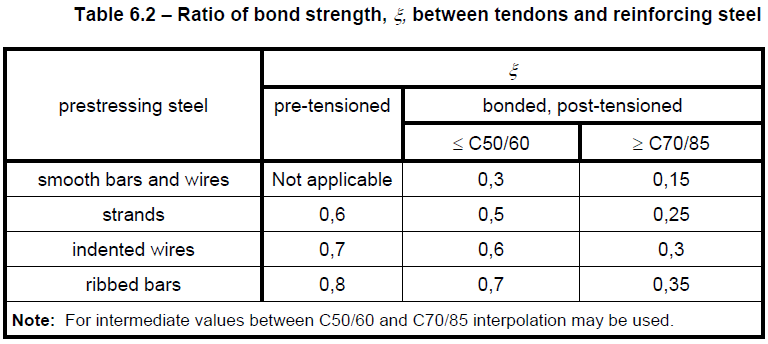The effective area of concrete in tensionAn important but simultaneously the most complicated step of the calculation is determining the effective area of the tensile concrete surrounding the reinforcement. Both the Eurocode and the Model Code consider simple modes of loading, where the reinforced concrete element is loaded by uniaxial bending or tension. The value of the effective height is determined as:${{h}_{c,eff}}=\min \left\{ 2,5\left( h-d \right);\frac{\left( h-x \right)}{3};{}^{h}/{}_{2} \right\}$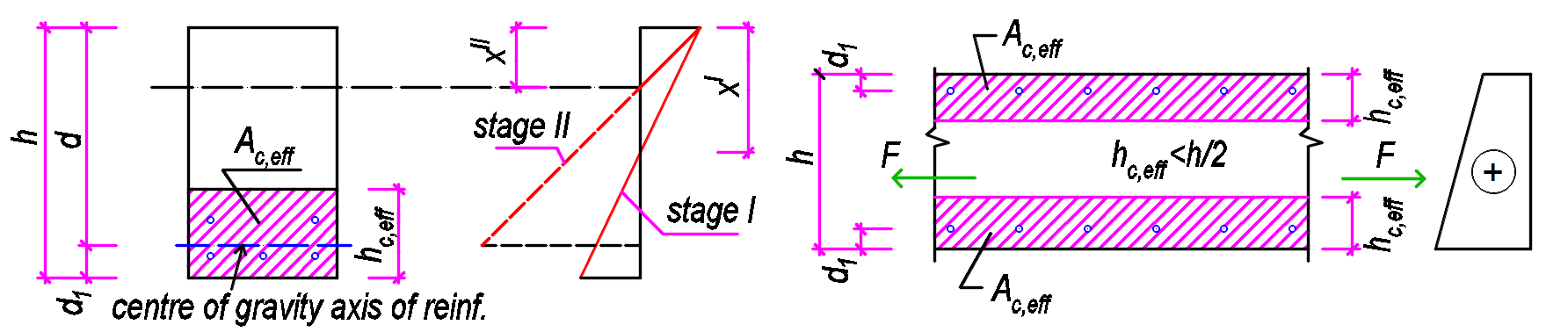$\textsf{\textit{\footnotesize{Fig. 6 Determination of Ac,eff for bent members (left) and members in tension (right)}}}$Usually, the value hc,eff = 2,5(h-d) is critical. For tensioned elements, the upper limit is h/2, while for bent elements it is (h-x)/3. However, the area Ac,eff is also limited by the width determined from equation 5(c+ϕ/2). If the spacing of the reinforcements is greater than 5(c+ϕ/2), then the effective area of the tensioned concrete of width 5(c+ϕ/2) is considered for the individual bars.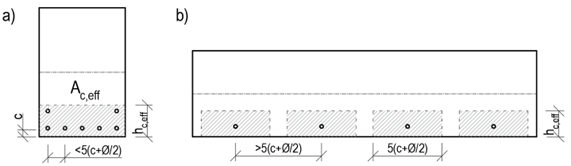$\textsf{\textit{\footnotesize{Fig. 9 Determination of Ac,eff based on reinforcement spacing}}}$Maximum crack distanceWhen calculating the maximum crack distance sr,max, two cases can occur: The axial distance of the bonded reinforcement does not exceed a distance of 5(c+ϕ/2) - Fig. 9a The axial distance of the bonded reinforcements is greater than 5(c+ϕ/2) - Fig. 9b The calculation of the maximum crack distance sr,max for the case that the axial distances of the reinforcements do not exceed the value 5(c+ϕ/2) is defined as follows:${{s}_{r,\max }}={{k}_{3}}c+{{k}_{1}}{{k}_{2}}{{k}_{4}}\frac{\phi }{{{\rho }_{p,eff}}}$c . . . . . concrete cover value in mm. Since the cover value may be different for the edge reinforcement to both the horizontal and vertical edges, it is recommended to consider the maximum cover value found for the reinforcement under consideration.ϕ . . . . diameter of the bonded reinforcement. In the case of different reinforcement diameters, the equivalent diameter shall be calculated in accordance with EN 1992-1-1 Equation 7.12.${{\phi }_{eq}}=\frac{{{n}_{1}}\phi _{1}^{2}+{{n}_{2}}\phi _{2}^{2}}{{{n}_{1}}{{\phi }_{1}}+{{n}_{2}}{{\phi }_{2}}}$k1 . . . . is a coefficient that takes account of the bond properties of the bonded reinforcement k1 = 0,8 for high bond bars k1 = 1,6 for bars with an effectively plain surface (e.g. prestressing tendons) k2 . . . . is a coefficient that takes account of the distribution of strain k2 = 1,0 for bending k2 = 0,5 for pure tension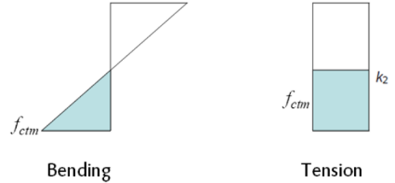For cases of eccentric tension or for local areas, intermediate values of k2 should be used which may be calculated from the relation:${{k}_{2}}=\frac{{{\varepsilon }_{1}}+{{\varepsilon }_{2}}}{2{{\varepsilon }_{1}}}$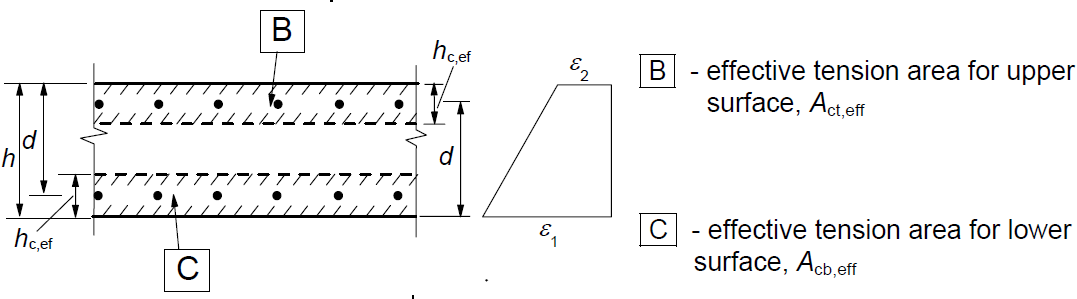k3 . . . . coefficient expressing the length of the area close to a crack where the bond between the concrete and the reinforcement is broken. The recommended value of the basic EC k3 = 3,4 may be modified by the National Annex. k4 . . . . coefficient expresses the relationship between the bond and tensile strength of concrete. The recommended value of the basic EC k4 = 0.425 may be adjusted by the National Annex.The calculation of the maximum crack distance sr,max for the case that the axial distances of the reinforcements exceed the value 5(c+ϕ/2) is defined as follows:${{s}_{r,\max }}=1,3\left( h-x \right)$Maximum crack distance values according to the equation${{s}_{r,\max }}=1,3\left( h-x \right)$should always be greater than the values determined by the equation${{s}_{r,\max }}={{k}_{3}}c+{{k}_{1}}{{k}_{2}}{{k}_{4}}{\phi }/{{{\rho }_{p,eff}}}\;$otherwise, it is recommended to consider the larger distance obtained from the above equations. The equation for the strain in the concrete/reinforcement is not modified for the case of the large axial distance of the reinforcement. In areas with controlled crack widths, the axial distance of individual reinforcements should not be greater than 5(c+ϕ/2).Crack width calculation implemented in RCSDetermination of effective area Ac,effBecause it is not so straightforward to determine which reinforcement can be considered as longitudinal crack-resisting reinforcement, Ac,eff is determined by using the following iterative process. Of all the reinforcement acting in tension, the tensile force center Cg,s,1 is determined. The effective depth of the reinforcement d is the distance between Cg,s, and the most compressed concrete fiber calculated in the direction of the resultant bending moment. At the same time, the position of the neutral axis and the height of the compressed area x for the cracked cross-section are determined. This makes it possible to determine the effective height hc,eff: ${{h}_{c,eff}}=\min \left\{ 2,5\left( h-d \right);\frac{\left( h-x \right)}{3};{}^{h}/{}_{2} \right\}$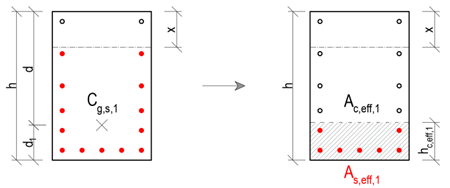By excluding all reinforcement that lie outside the Ac,eff,1, the new center of the reinforcement Cg,s,2 is determined, together with the new effective depth of the reinforcement d, effective height hc,eff is determined in the same way as in the previous step, only with changed input values.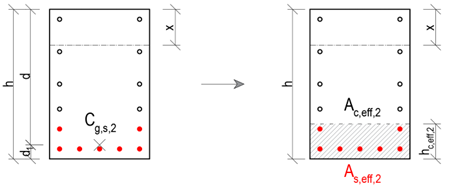Again, it is checked that all the tensioned reinforcement under consideration lies in the Ac,eff,2. If this condition is satisfied, the iteration can be terminated and the values of hc,eff,2, Ac,eff,2 and As,eff,2 are displayed as the resulting values in IDEA StatiCa RCS.Possible cases of crack width calculationIn general, three cases can occur when calculating crack widths: The tensile reinforcement lies in the region Ac,eff, with the axial distance of the individual reinforcements being less than 5(c+ϕ/2). Then the following definitions are used for the calculation: ${{s}_{r,\max }}={{k}_{3}}c+{{k}_{1}}{{k}_{2}}{{k}_{4}}\frac{\phi }{{{\rho }_{p,eff}}}$${{\varepsilon }_{sm}}-{{\varepsilon }_{cm}}=\frac{{{\sigma }_{s}}-{{k}_{t}}\,\cdot \,\frac{{{f}_{ct,eff}}}{{{\rho }_{p,eff}}}\,\cdot \,\left( 1+\,{{\alpha }_{e}}\cdot \,{{\rho }_{p,eff}} \right)\,\,}{{{E}_{s}}}\ge 0,6\frac{{{\sigma }_{s}}}{{{E}_{s}}}$ The tensile reinforcement lies in the Ac,eff, with the axial distance of the individual reinforcements exceeding the distance 5(c+ϕ/2). Then the following definitions are used for the calculation: ${{s}_{r,\max }}=1,3\left( h-x \right)$${{\varepsilon }_{sm}}-{{\varepsilon }_{cm}}=\frac{{{\sigma }_{s}}-{{k}_{t}}\,\cdot \,\frac{{{f}_{ct,eff}}}{{{\rho }_{p,eff}}}\,\cdot \,\left( 1+\,{{\alpha }_{e}}\cdot \,{{\rho }_{p,eff}} \right)\,\,}{{{E}_{s}}}\ge 0,6\frac{{{\sigma }_{s}}}{{{E}_{s}}}$ The tensile reinforcement does not lie in the Ac,eff (this may be caused, for example, by thick cover).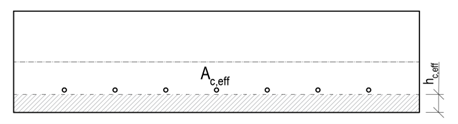In this case it would not be possible to calculate the width of the cracks. Therefore, the calculation of the effective height hc,eff is modified as follows:${{h}_{c,eff}}=\min \left\{ 2,5\left( h-d \right);h/2 \right\}$At the same time, the following nonconformity is displayed:The effective concrete area in tension surrounding the reinforcement or prestressing tendons of depth hc,eff, where hc,eff is the lesser of 2.5(h – d) or h/2. Considering value as (h – x)/3, the reinforcement is out of the effective area of concrete in tension, and therefore it would not be possible to calculate crack width according to clause 7.3.4. N-M-κ diagram The N-M-κ diagram shows an element's curvature (bending stiffness) as a function of an applied bending moment and normal force. There are three types of N-M-κ diagrams: - short-term, - long-term - ULS. These diagrams differ in the types of stress-strain diagrams used for the calculation (explained below).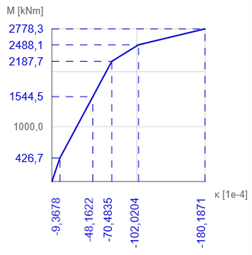The stiffness calculation for selected characteristic states of the cross-section is used to determine the N-M-κ diagram. In general, it can be any cross-sectional state from which the response is calculated and from which the bending stiffness and curvature are derived. In IDEA RCS, we consider four characteristic points (Mr, Mc, Ms and Mu)Mr - the cracking moment The cross-section is subjected to user-defined normal force and the plane of strain starts to rotate (in the direction of the specified bending moment) until the ultimate tensile strength of the concrete is reached in a concrete fibre (for concrete grade C30/37 this is fctm = 2,896 MPa). A bilinear stress-strain diagram with a horizontal plastic branch for both reinforcement and concrete is used for the calculation.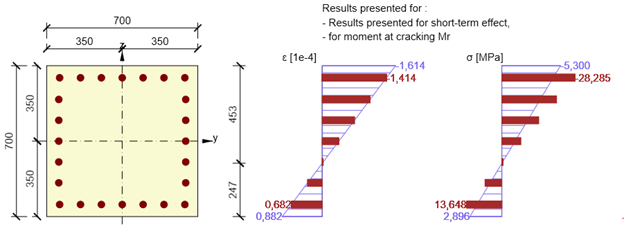Mc - the bending moment when the compressive strength of the concrete is reachedFrom the previous step, the most utilized concrete fibre in compression is identified. For this fibre, the strain at the ultimate strength of concrete (fck/Ecm for short-term, fck/Eceff for long-term and fcd/Ecm for the ULS diagram) is set. Based on the defined normal force and the direction of bending moment, the iteration process to find the plane of strain is run to find an equilibrium between the response of the cross-section and the defined normal force. A bilinear stress-strain diagram with a horizontal plastic branch for both reinforcement and concrete is used for the calculation.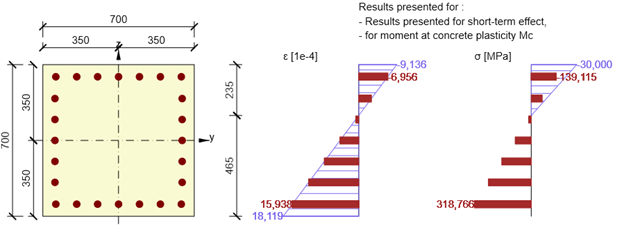Ms - the bending moment when the yield strength in the most utilized reinforcement bar is reachedAnother characteristic point of the N-M-κ diagram is the stress-state of the cross-section when the yield strength in the most utilized reinforcement bar is reached (rebar strain is equal to fyk/Es for the short- and long-term diagrams, fyd/Es for the ULS diagram). The iteration process finds an equilibrium of normal forces in the cross-section by rotating the plane of strain around the point specified by the position of the most utilized reinforcement bar. A bilinear stress-strain diagram with a horizontal plastic branch for both reinforcement and concrete is used for the calculation.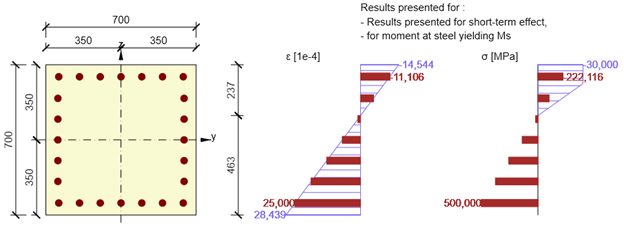Mu - the bending moment at the ultimate limit stateThis is the ultimate load bearing capacity of a cross-section in bending, when the cross-section is subjected to defined design normal force Ned. For the calculation of the cross-sectional capacity it is assumed that the compressive strength in the most utilized fibre of concrete and the tensile strength in most utilized reinforcement bar are reached (maximum strain for concrete εcu = 0,1 and for reinforcement εs,max = 0,5. A bilinear stress-strain diagram with a horizontal plastic branch for the reinforcement and a parabola-rectangular diagram for the concrete are used for the calculation.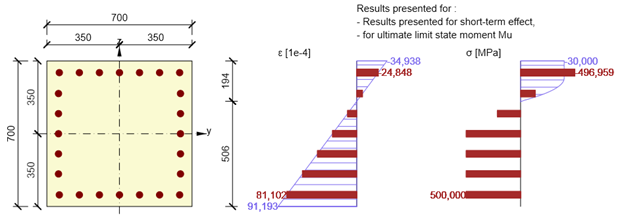The resulting stiffness and curvature due to the user-defined combination of normal force and bending moment ( Md) are then calculated using linear interpolation of the individual characteristic points of the N-M-κ diagram.Calculation of stiffnesses and curvaturesThe stiffnesses and curvatures for each cross-sectional stress state (Mr, Mc, Ms or Mu)are calculated directly from the rotation of the plane of strain. $E{{A}_{x}}=\frac{N}{{{\varepsilon }_{x}}}$EAx . . axial stiffness of the elementN . . . . the specified normal forceεx . . . axial strain at the centre of gravity of the concrete cross-section$E{{I}_{y}}=\frac{M}{\kappa }$EIy . . . bending stiffness of the elementM . . . the calculated bending moment Mr, Mc, Ms or Muκ . . . . the curvature of the element, calculated as the tangent of the angle between the plane of the strain and the longitudinal element axisPractical exampleA concrete cross-section (concrete grade C30/37) is reinforced with ϕ32 reinforcement (grade B500B). The defined quasi-permanent combination is N = -730 kN and My = 557 kNm.The plane of strain for the characteristic point Ms is determined by IDEA RCS as follows: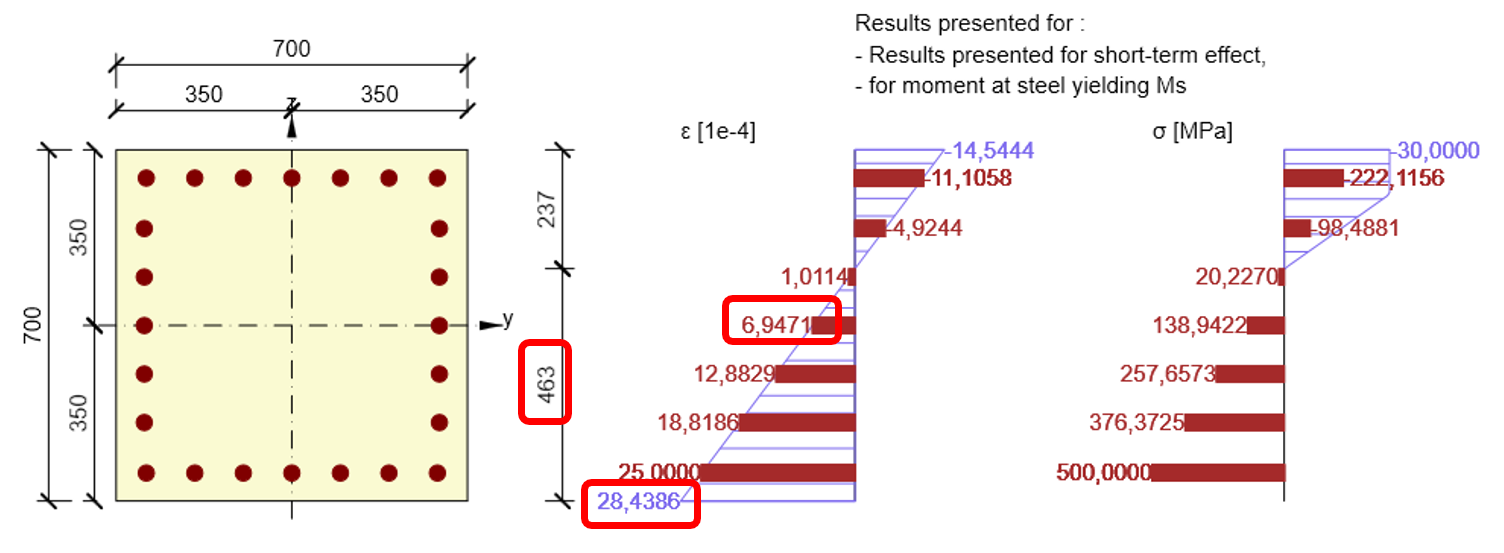$E{{A}_{x}}=\frac{N}{{{\varepsilon }_{x}}}=\frac{730}{6,9471\cdot {{10}^{-4}}}=1050,798MN$$\kappa =\frac{28,4386\cdot {{10}^{-4}}}{0,463}=61,422\cdot {{10}^{-4}}{{m}^{-1}}$$E{{I}_{y}}=\frac{{{M}_{s}}}{\kappa }=\frac{2277,4}{61,422\cdot {{10}^{-4}}}=370,776MN{{m}^{2}}$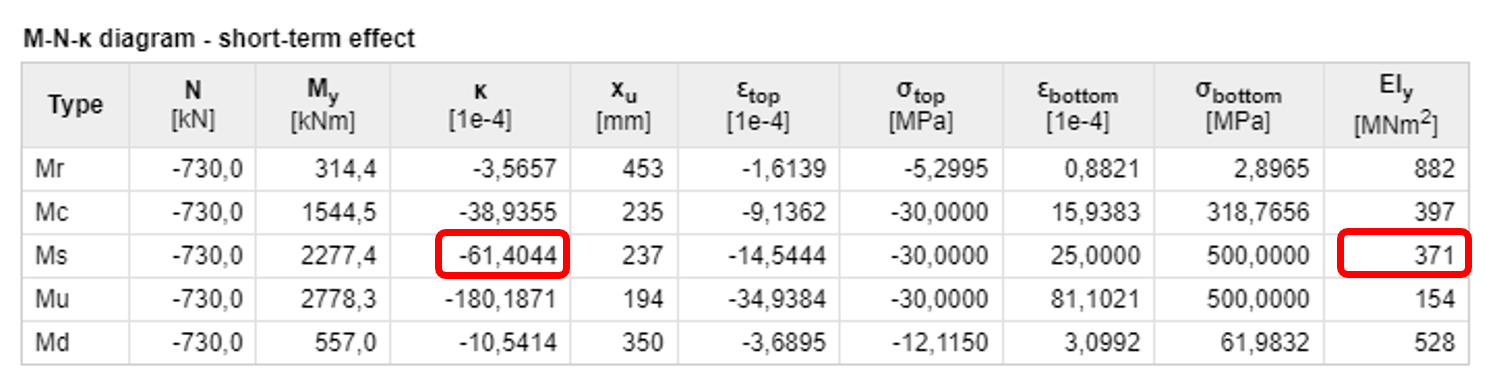Stress-strain diagrams used for the calculationReinforcement - Mr, Mc, Ms and Mu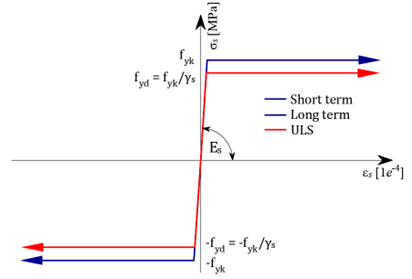Concrete - Mr, Mc, Ms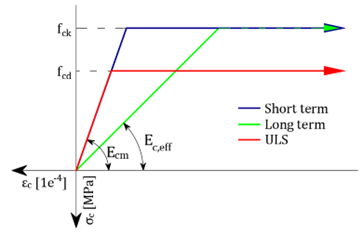Concrete - Mu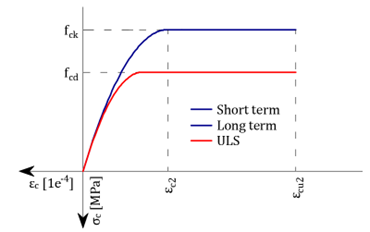Literature  Bradáč Betonové konstrukce (concrete structures), 1.part: Dimensioning of members from reinforced and plainconcrete, EXPERT Ostrava, 1996 ČSN EN 1992-1-1 (73 1201) Eurocode 2: Design of concrete structures - Part 1-1: General rules and rules for buildings, inc. change NA ed. A (2007) and revision 1 (2009) ČSN EN 1992-2 (73 6208) Eurokód 2: Navrhování betonových konstrukcí - Část 2: Betonové mosty - Navrhování a konstrukční zásady Navrátil, J. Předpjaté betonové konstrukce. 2. vydání, Akademické nakladatelství CERM, Vysoké učení technické v Brně, Fakulta stavební, 2008 Šmiřák, S. Pružnost a plasticita I, Vysoké učení technické v Brně, Akademické nakladatelství CERM, Brno, 1999 Vondráček, R. Numerical Methods in Nonlinear Concrete Design, Diplomová práce, ČVUT, Praha, 2000 Zich, M. a kolektiv Konstrukční Eurokódy - Příklady posouzení betonových prvků dle Eurokódů, on-line book http://www.stavebniklub.cz/konstrukcni-eurokody-onbecd/, Verlag Dashöfer, 2010 Try IDEA StatiCa for free Start your trial today and enjoy 14-days of full access and services free of charge. Start free trial$$